## Sunday, December 30, 2018

2015
(November)
MATHEMATICS
(Major)
Course: 502
(Linear Algebra and Number Theory)
Full Marks: 80
Pass Marks: 32
Time: 3 hours
The figures in the margin indicate full marks for the questions
GROUP - A
(Linear Algebra)
(Marks: 40)

1. (a) Write when a system of linear equations is said to be consistent. 1
(b) Show that the additive inverse of any vector in a vector space is unique. 2
(c) Find the solution set for the following system of equations: 3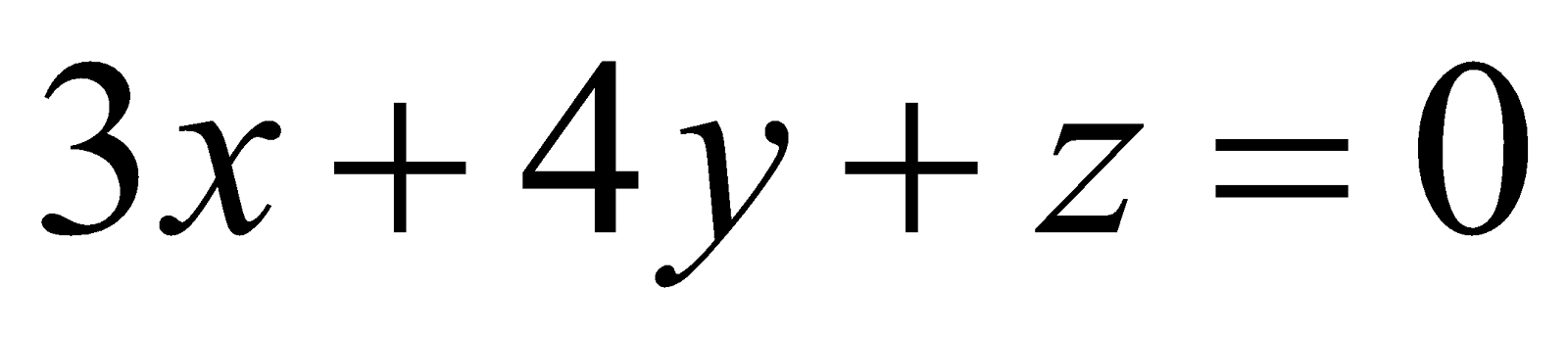And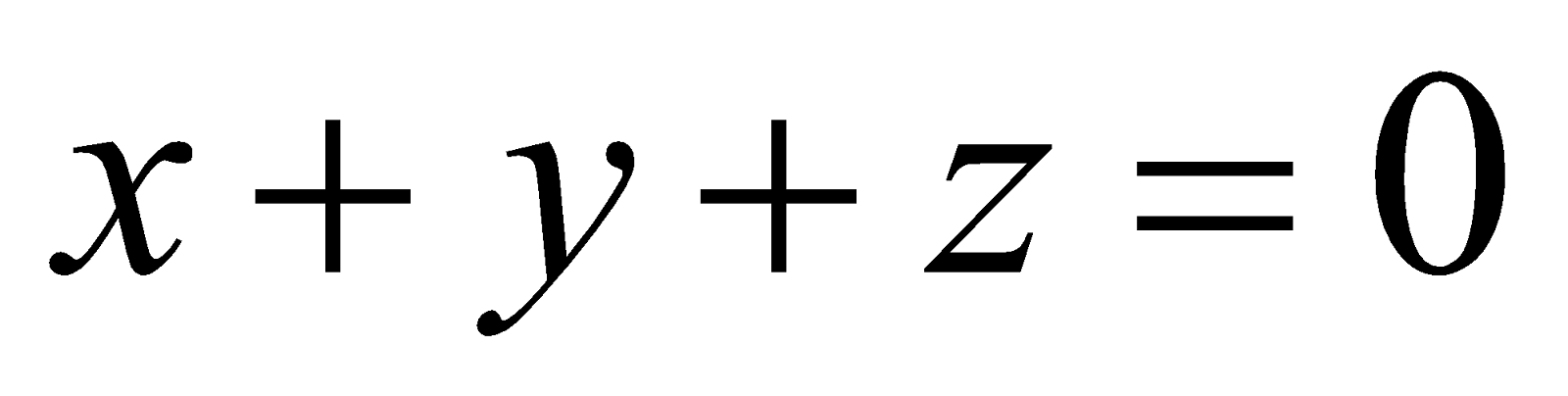2. Answer any two of the following:
1. Let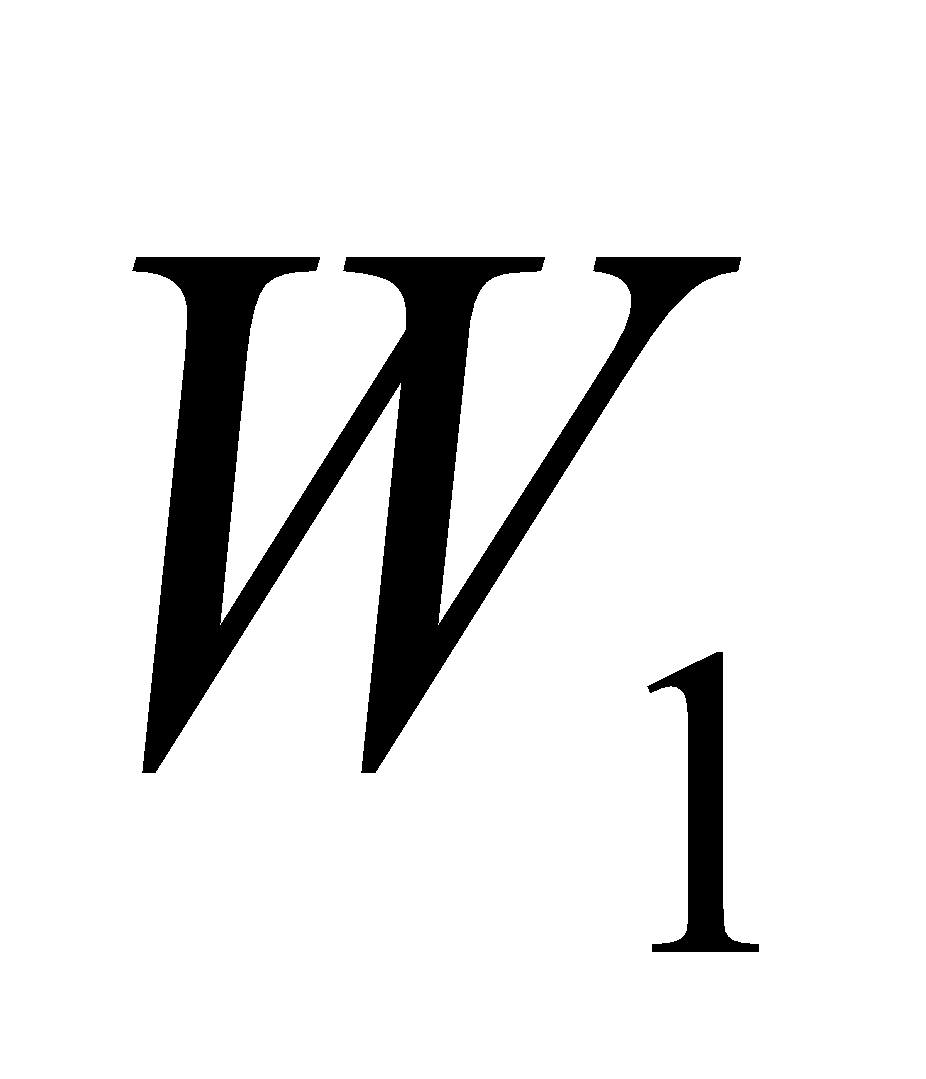and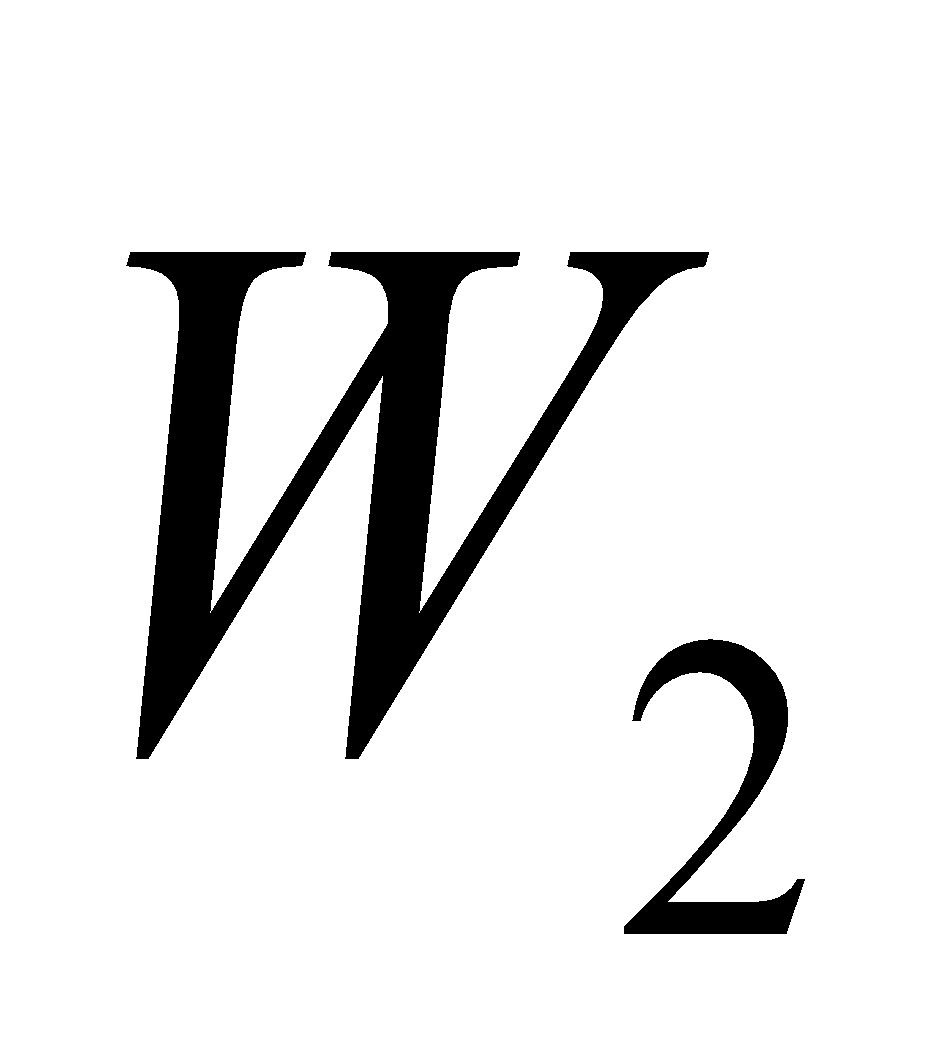be two subspaces of a vector space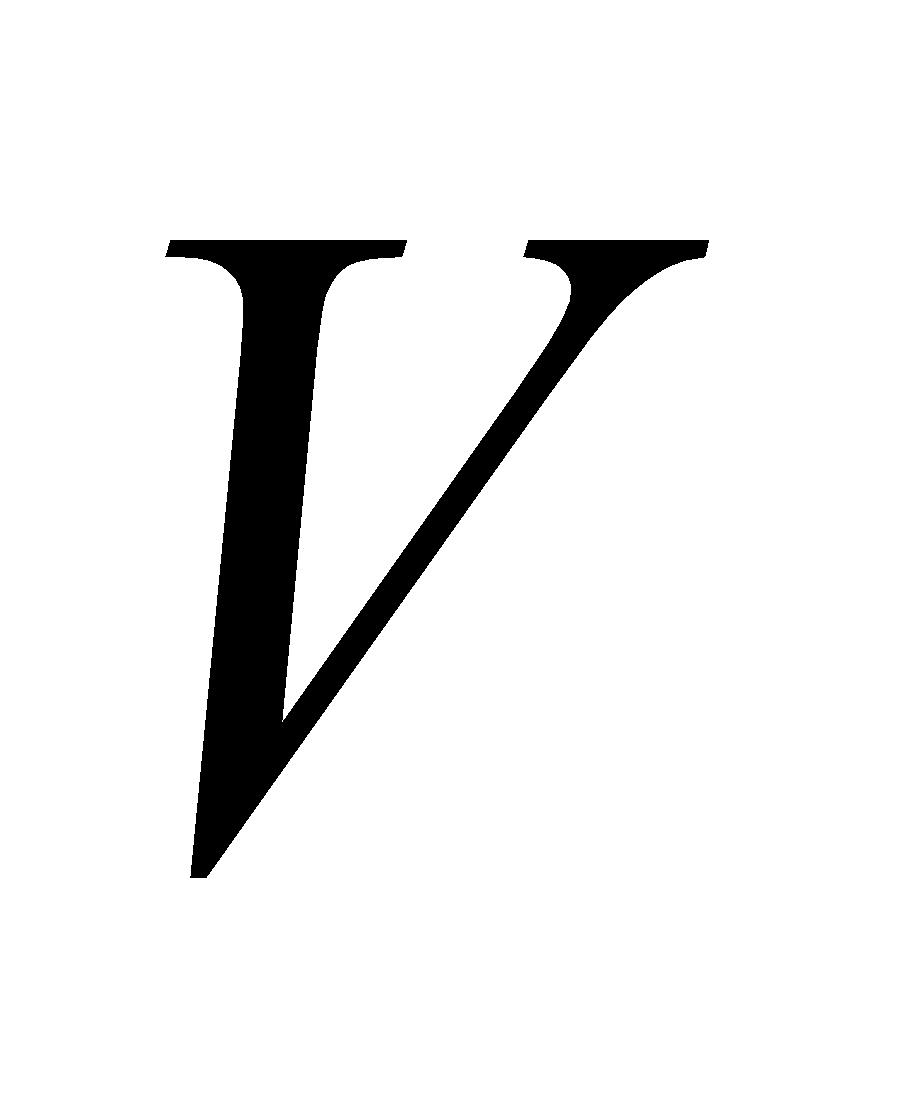over a field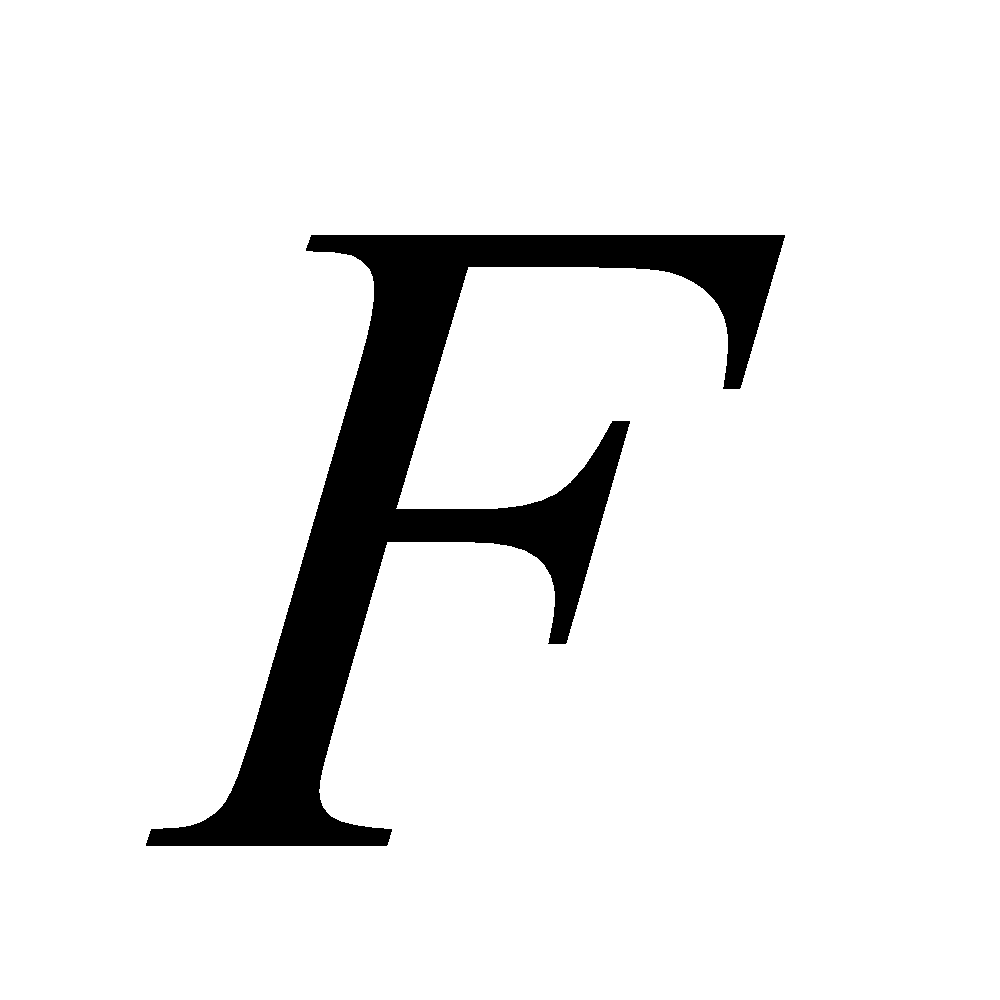. Show that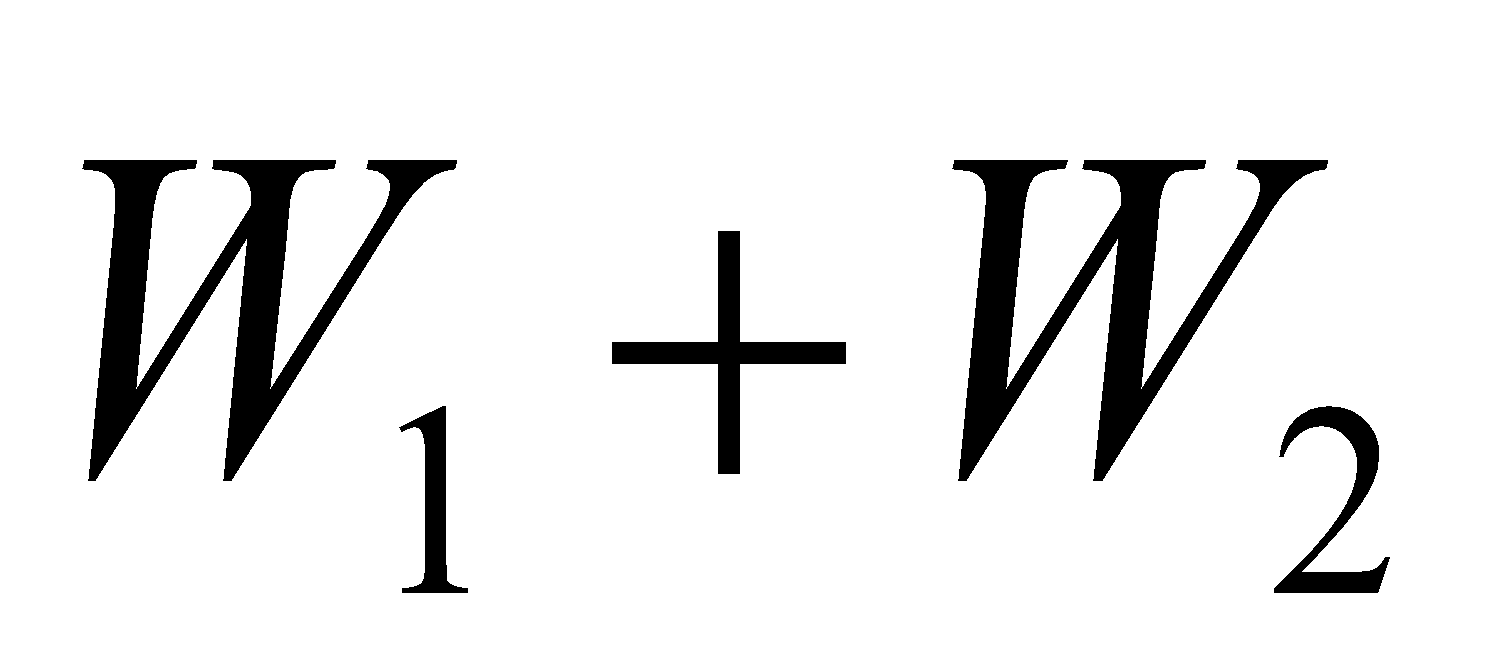is also a subspace of. 4
2. When is a set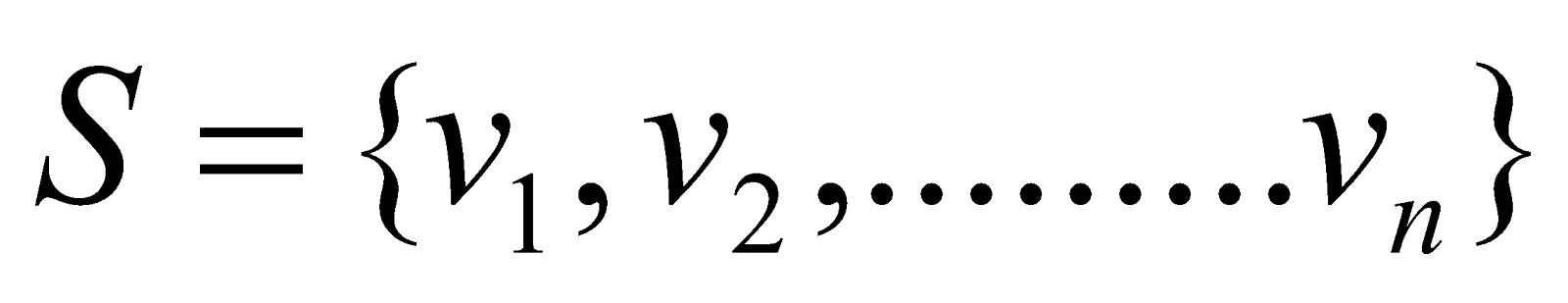of vectors in a vector spacesaid to be linearly independent? Examine whether the vectors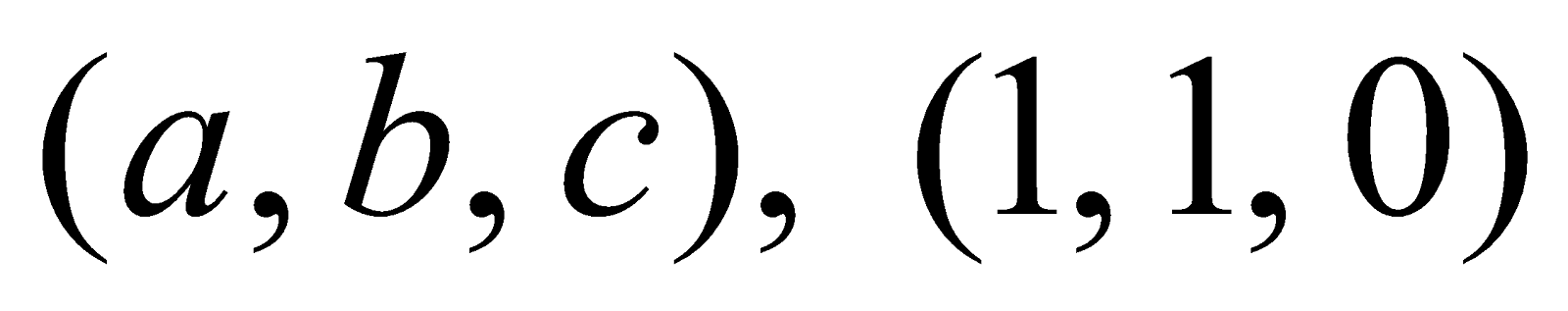and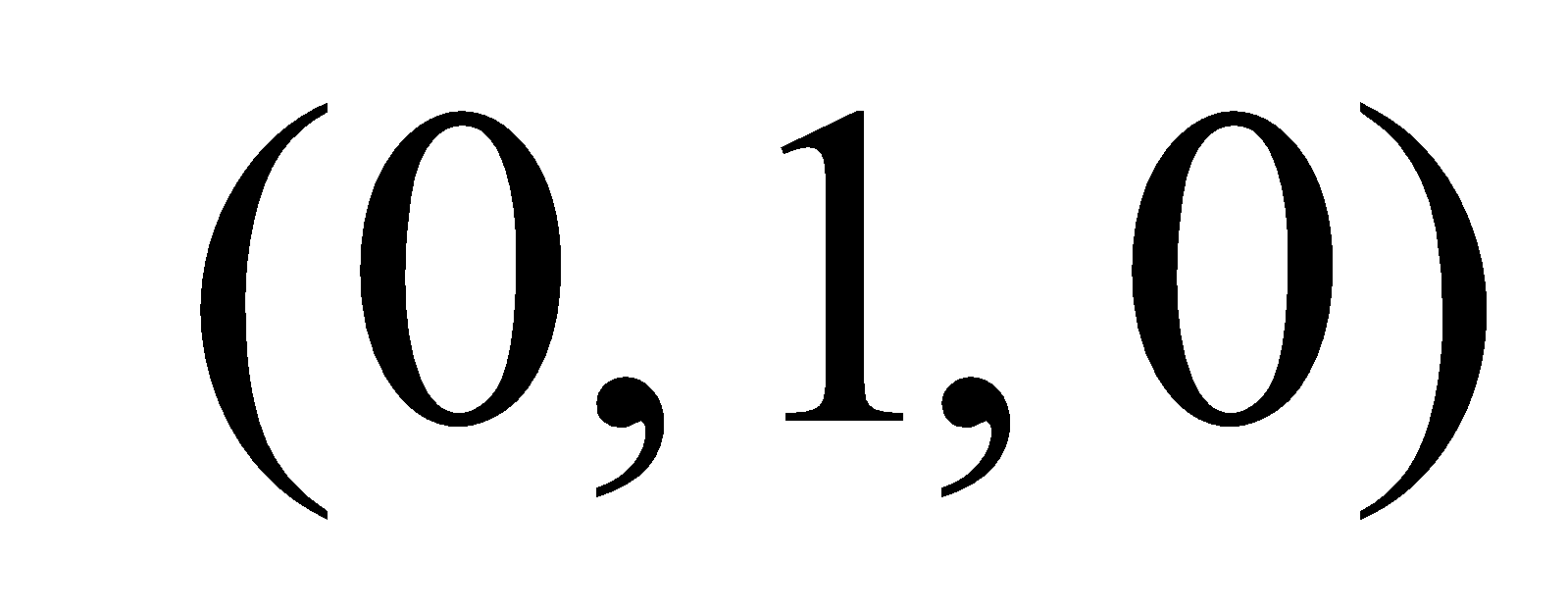of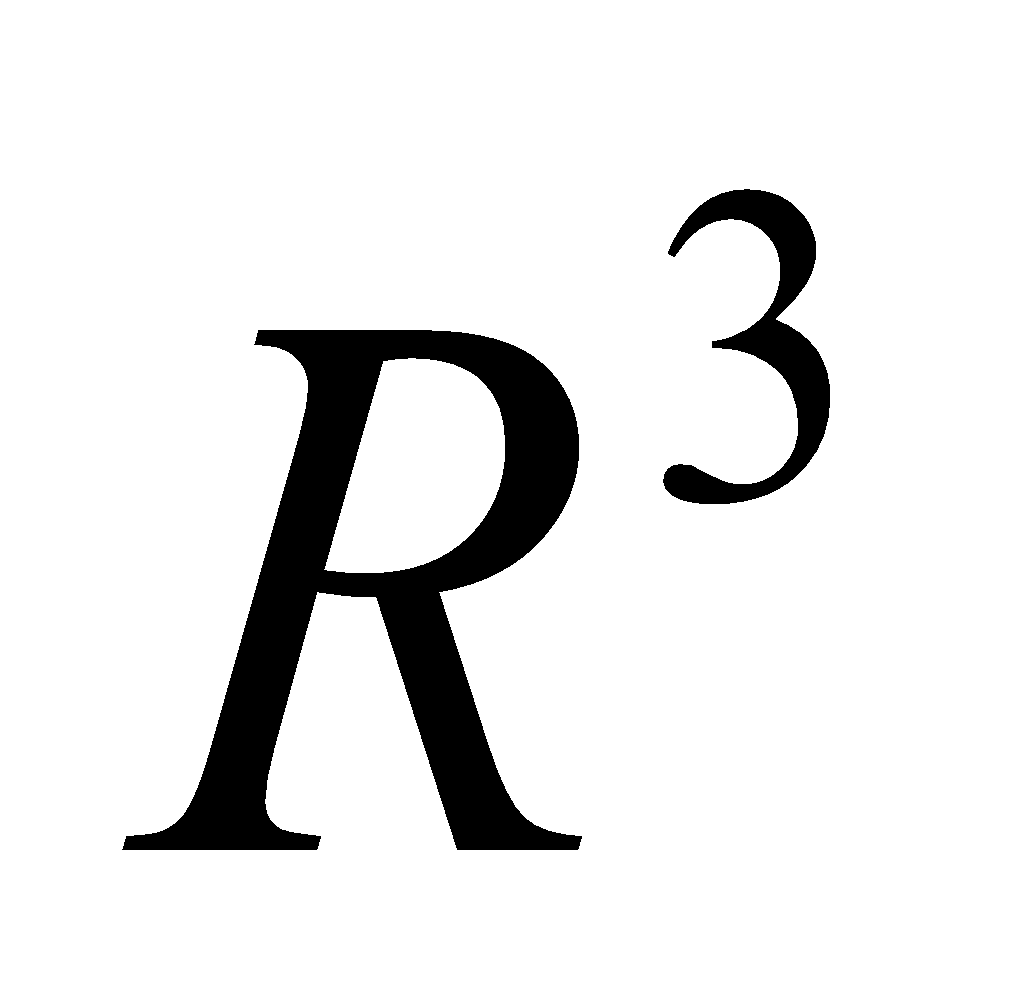are linearly independent over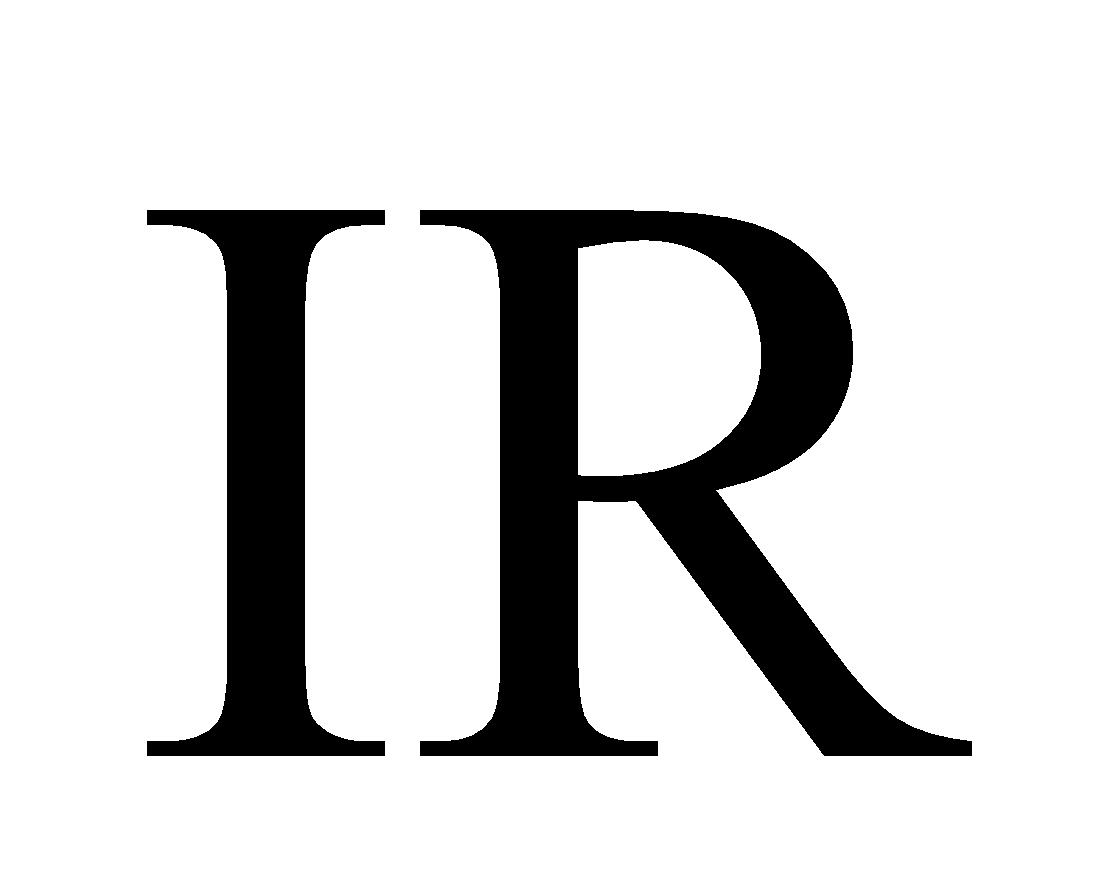, where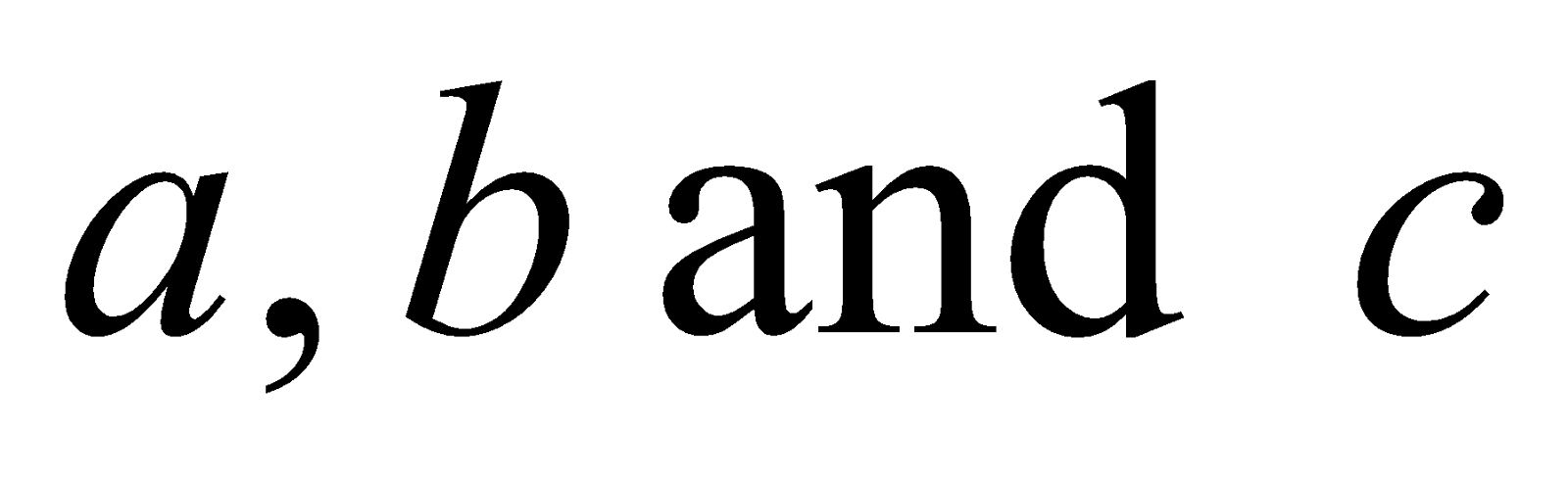are non-zero real numbers. 1+3=4
3. Show that the vectors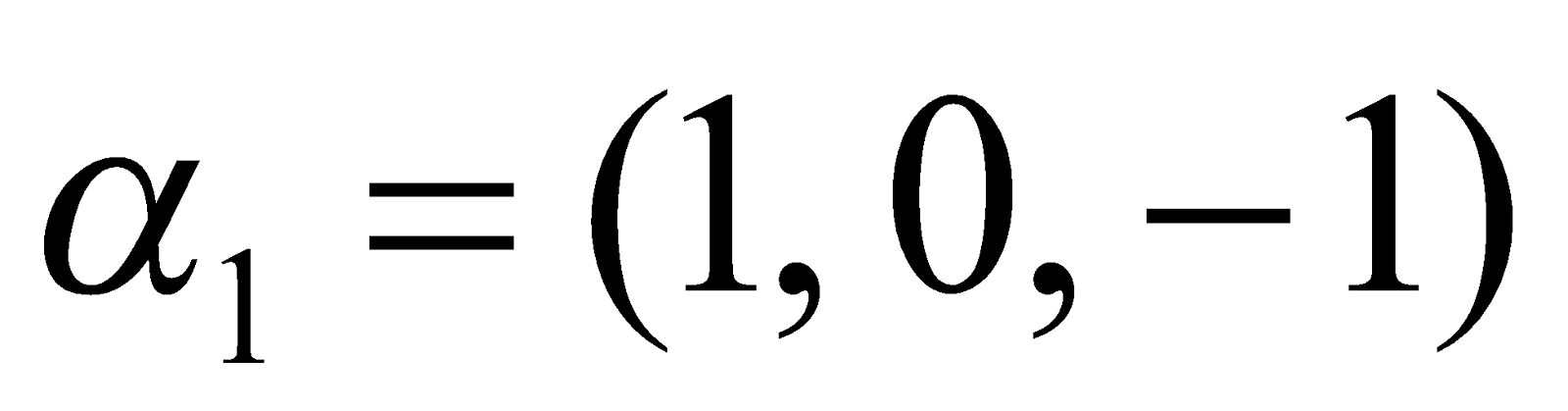,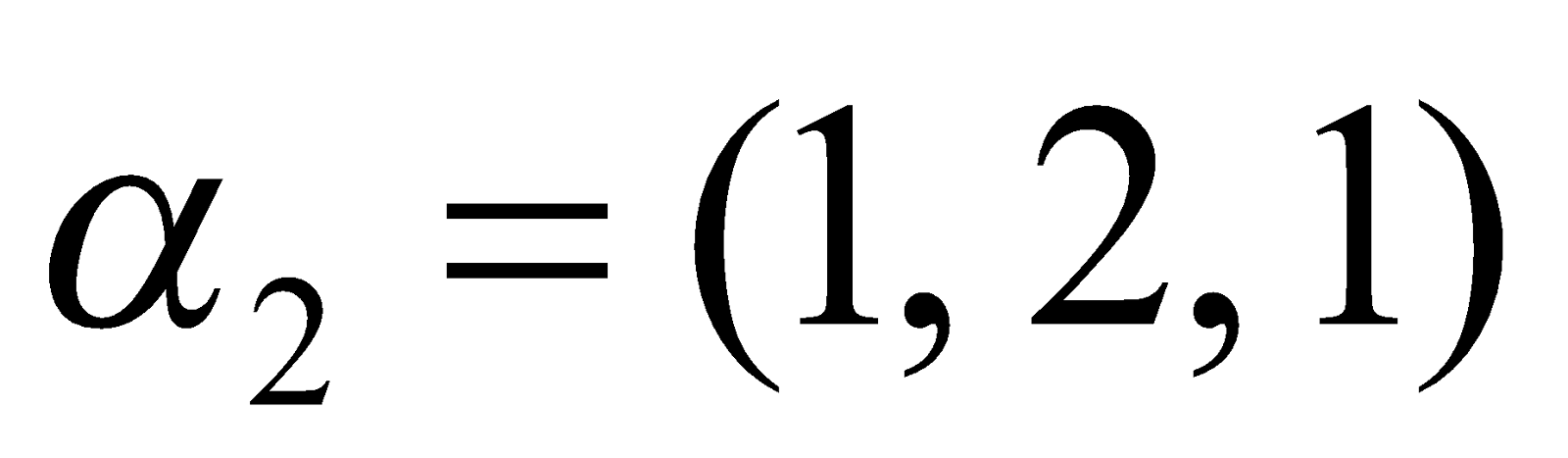andfrom a basis for
4
3. Define subspace of a vector space with an example. If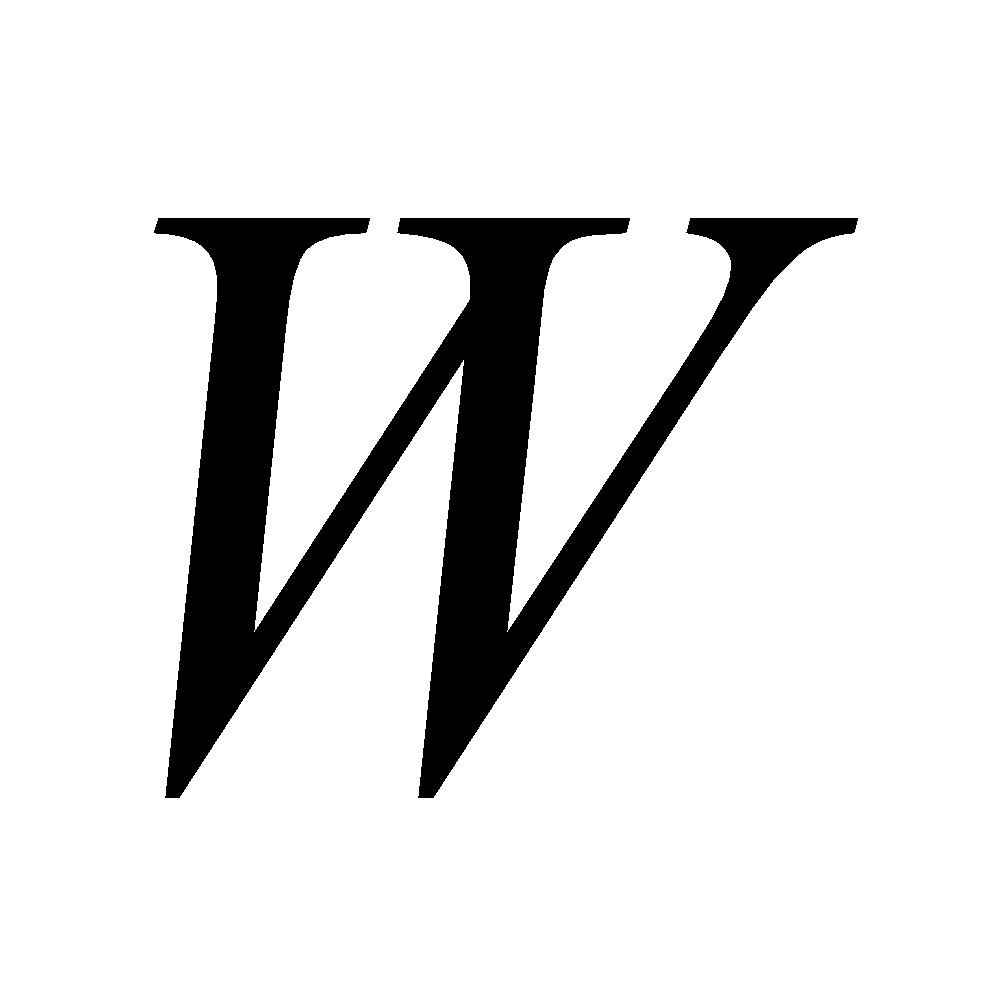is a proper subspace of a finite dimensional vector space, then prove thatis finite dimensional and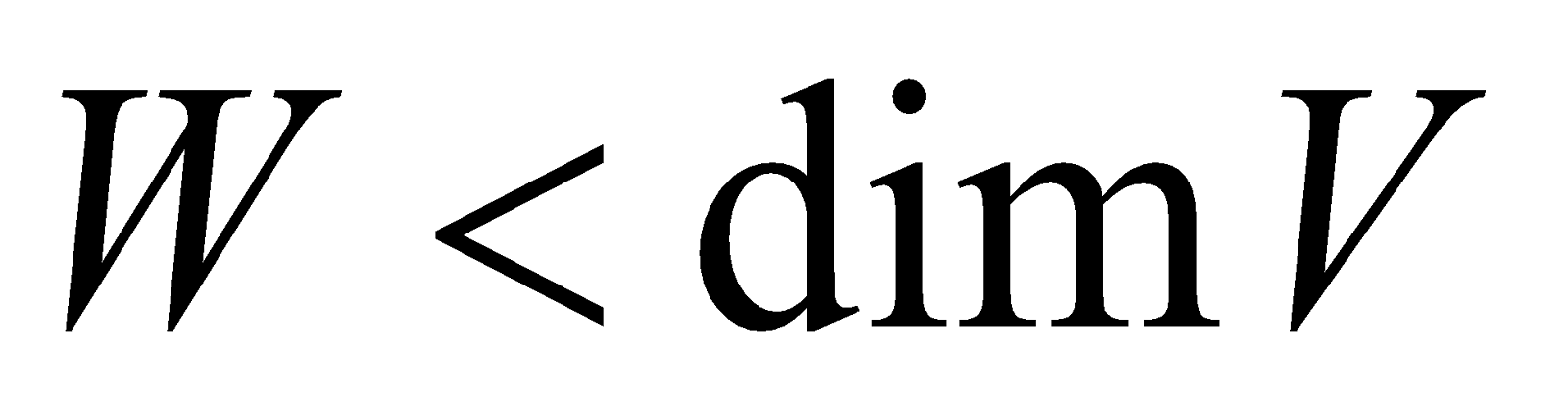. 2+3=5
4. Answer any three of the following:
1. Define a line in a vector space. Prove that any two distinct points determine a unique line in any vector space. 1+2=3
2. Letbe a vector subspace of a vector spaceand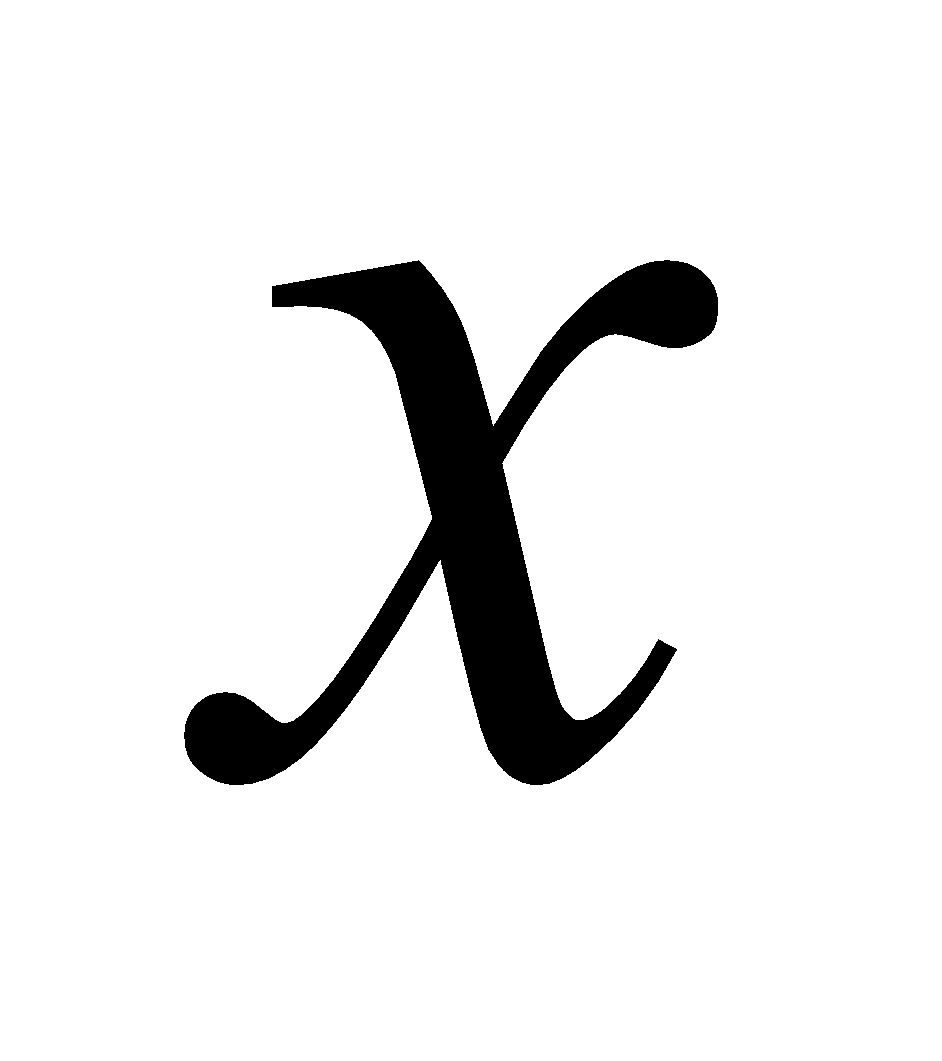be a fixed vector in. Prove that the set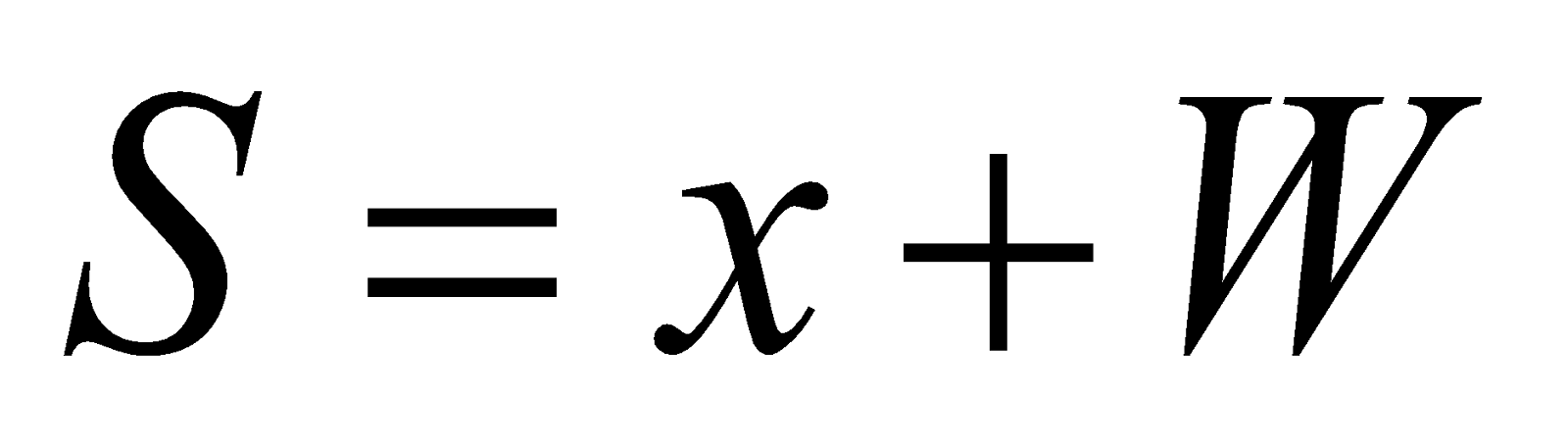is an affine space of. 3
3. Letbe a subspace of a vector spaceover a field. Show that two cosetsand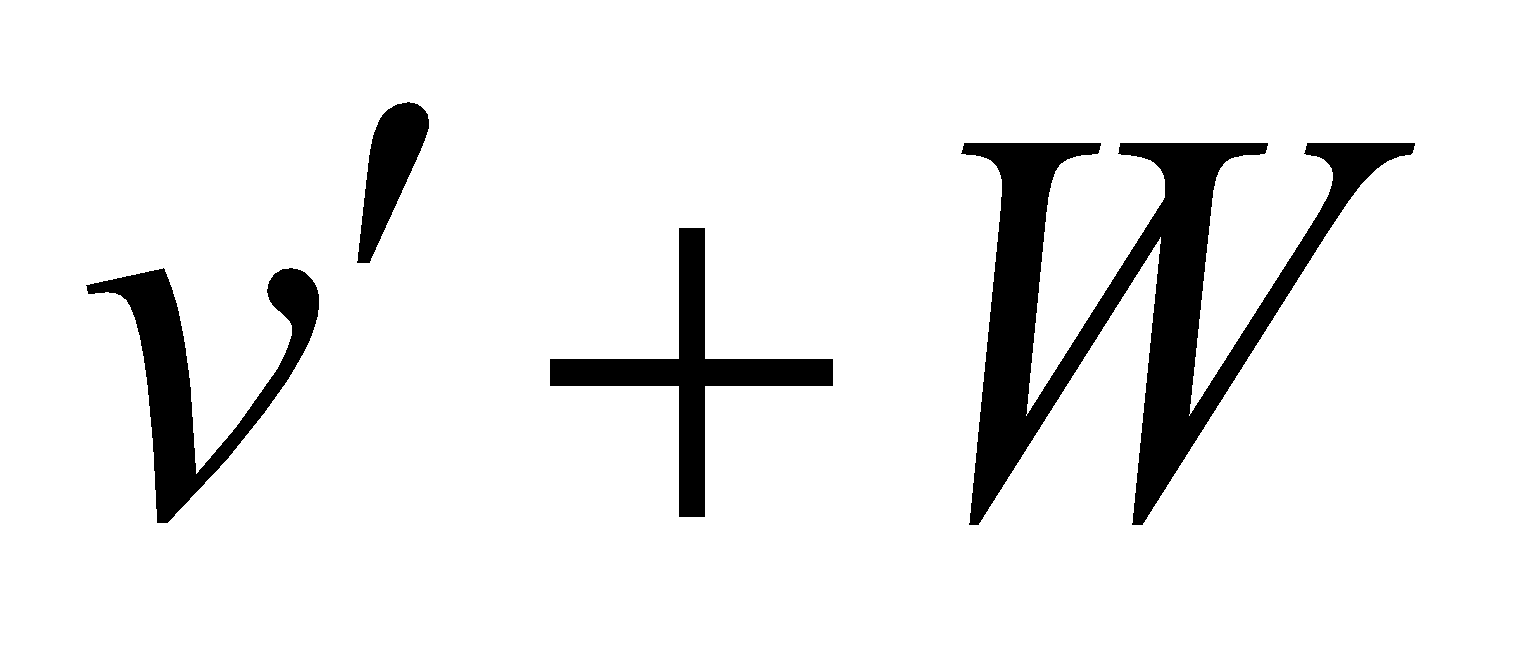are equal if and only if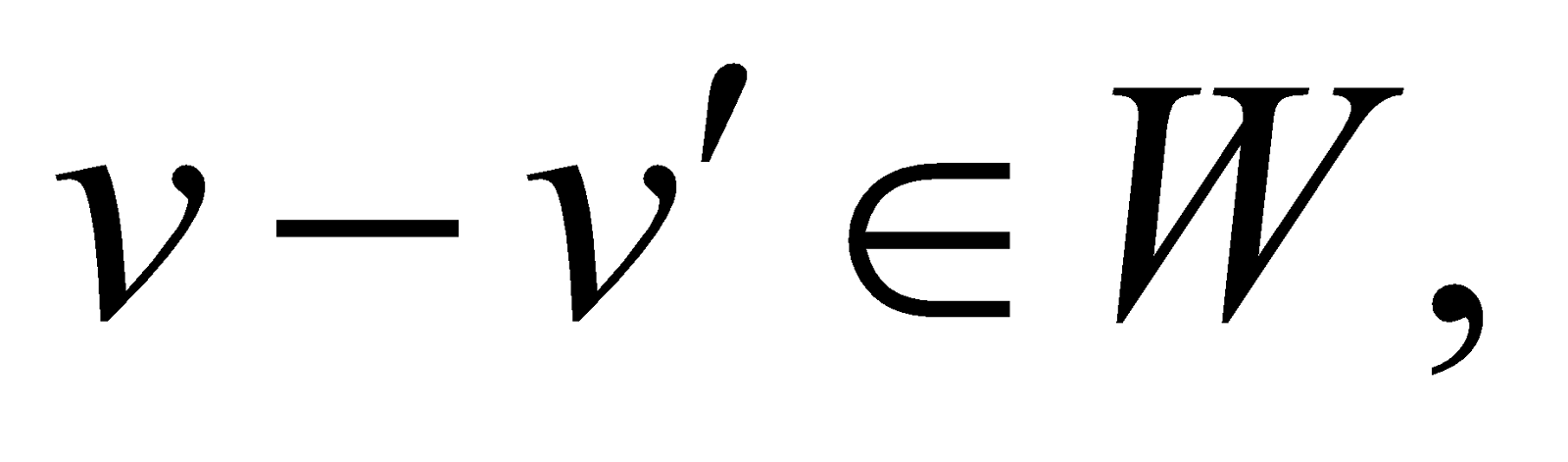where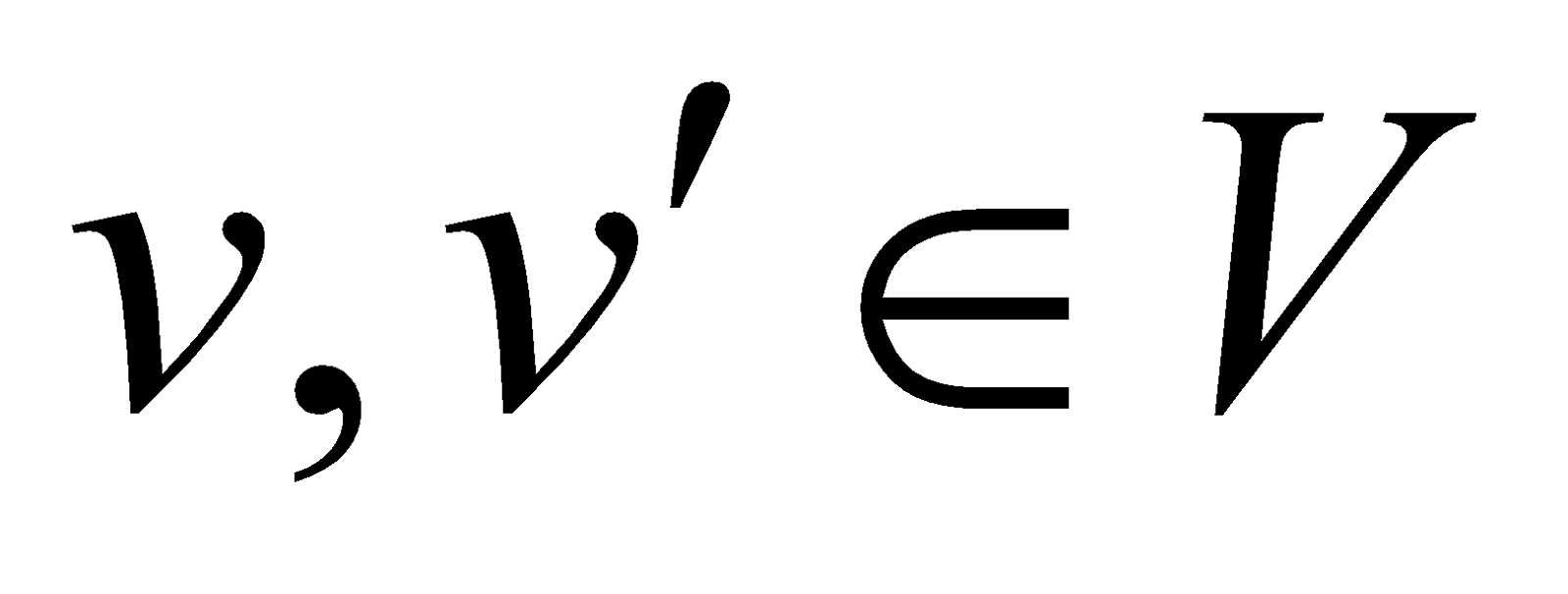. 3
4. Letbe the subspace of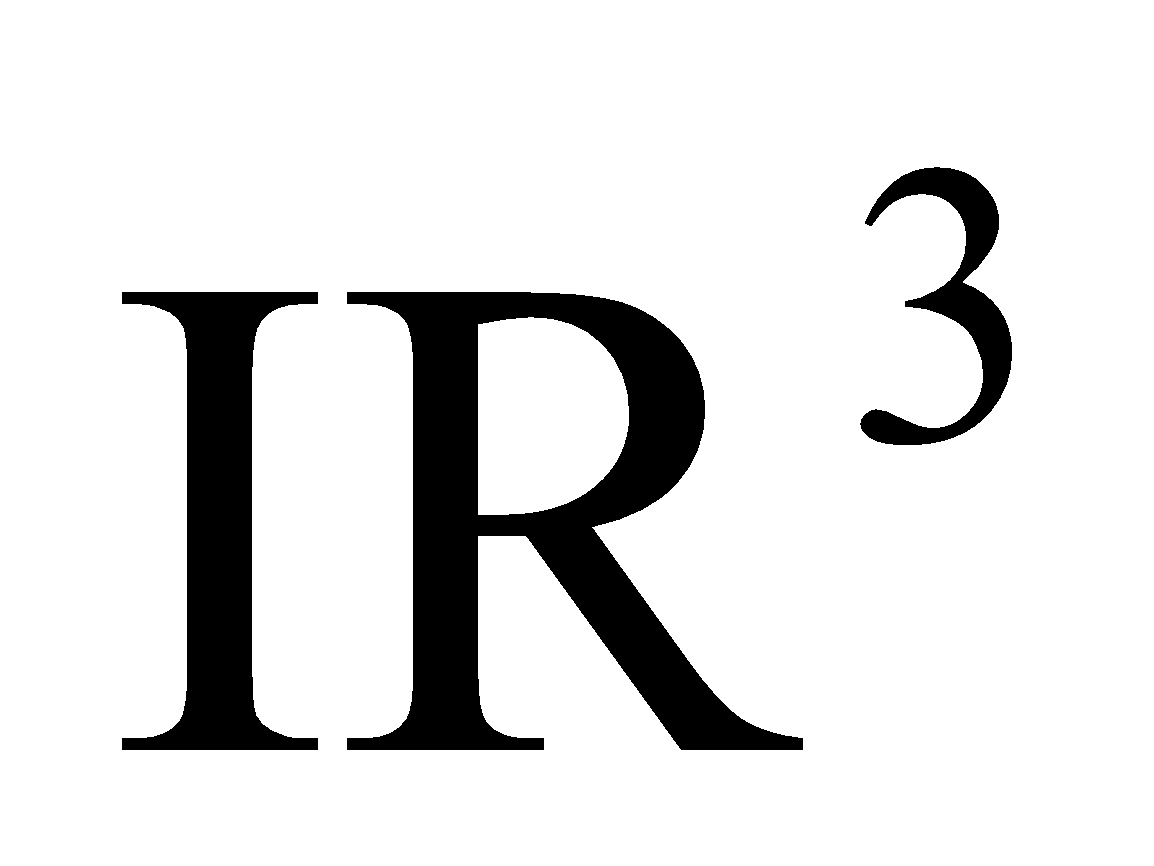spanned by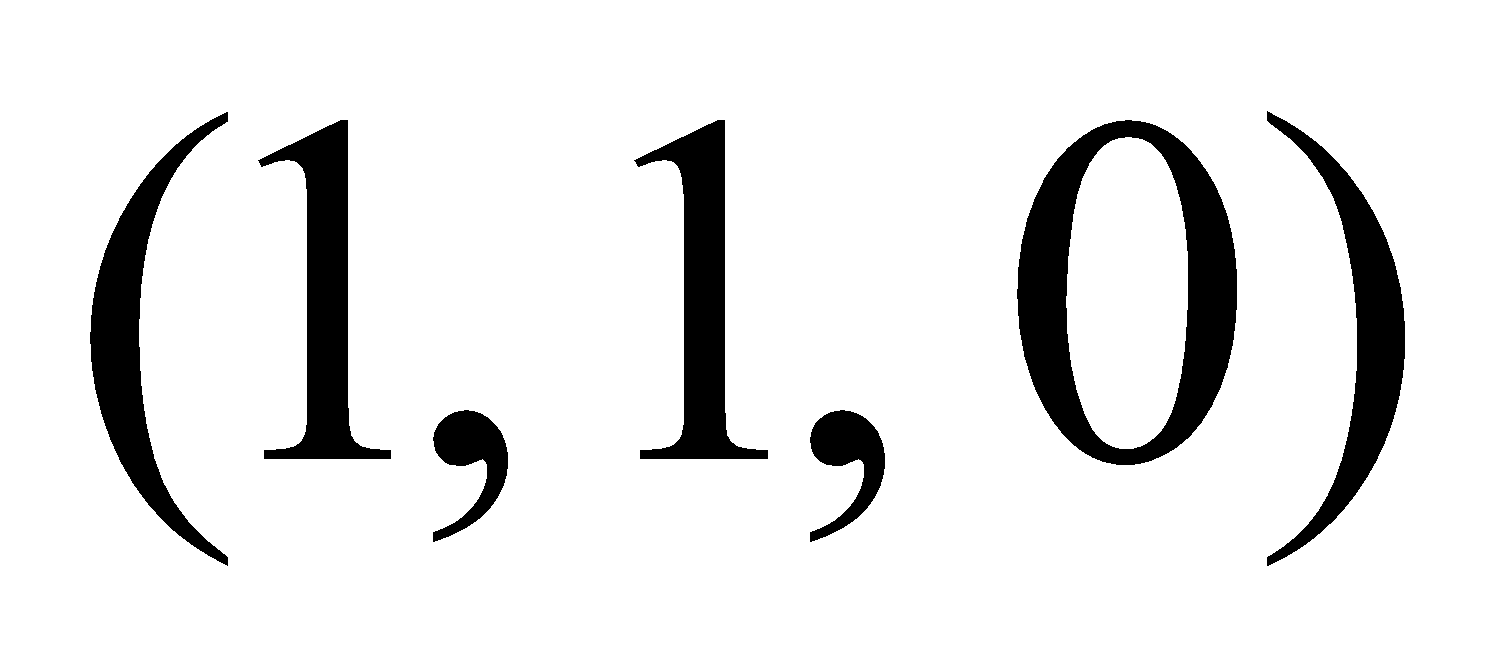and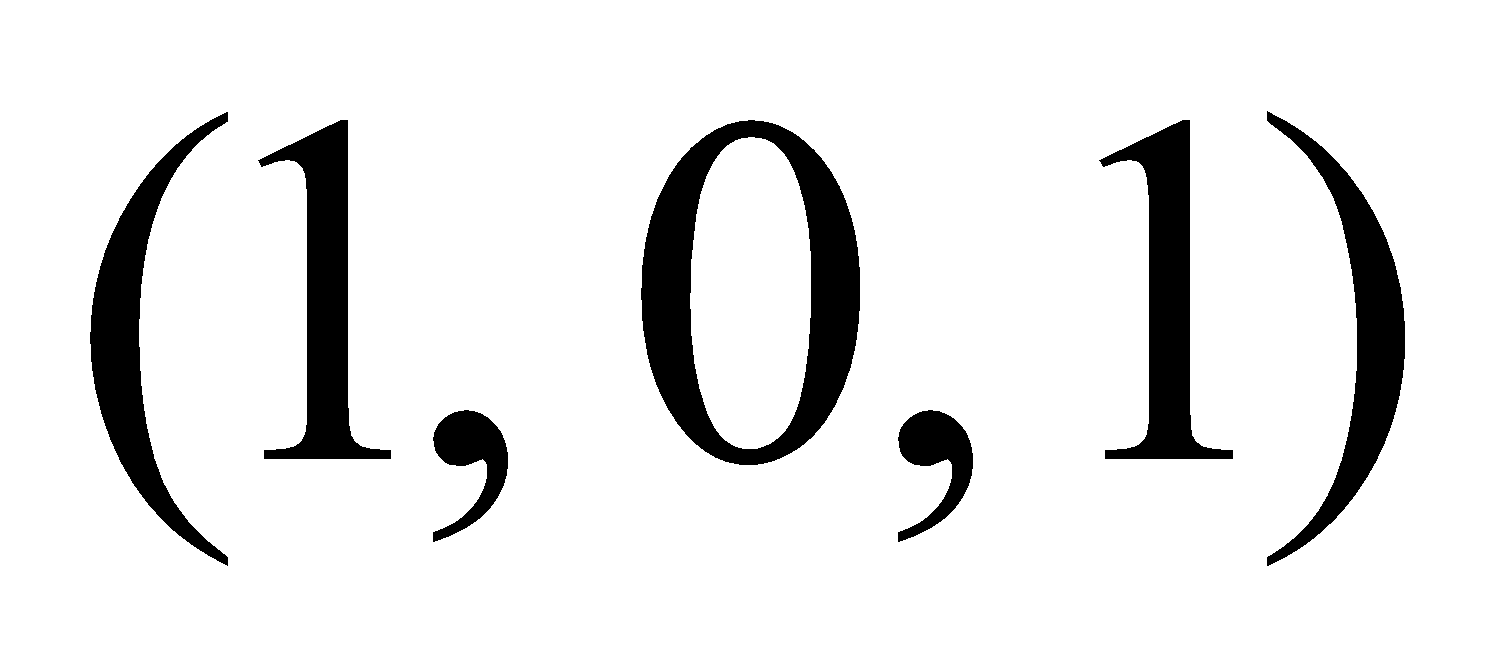. Find the basis for the quotient space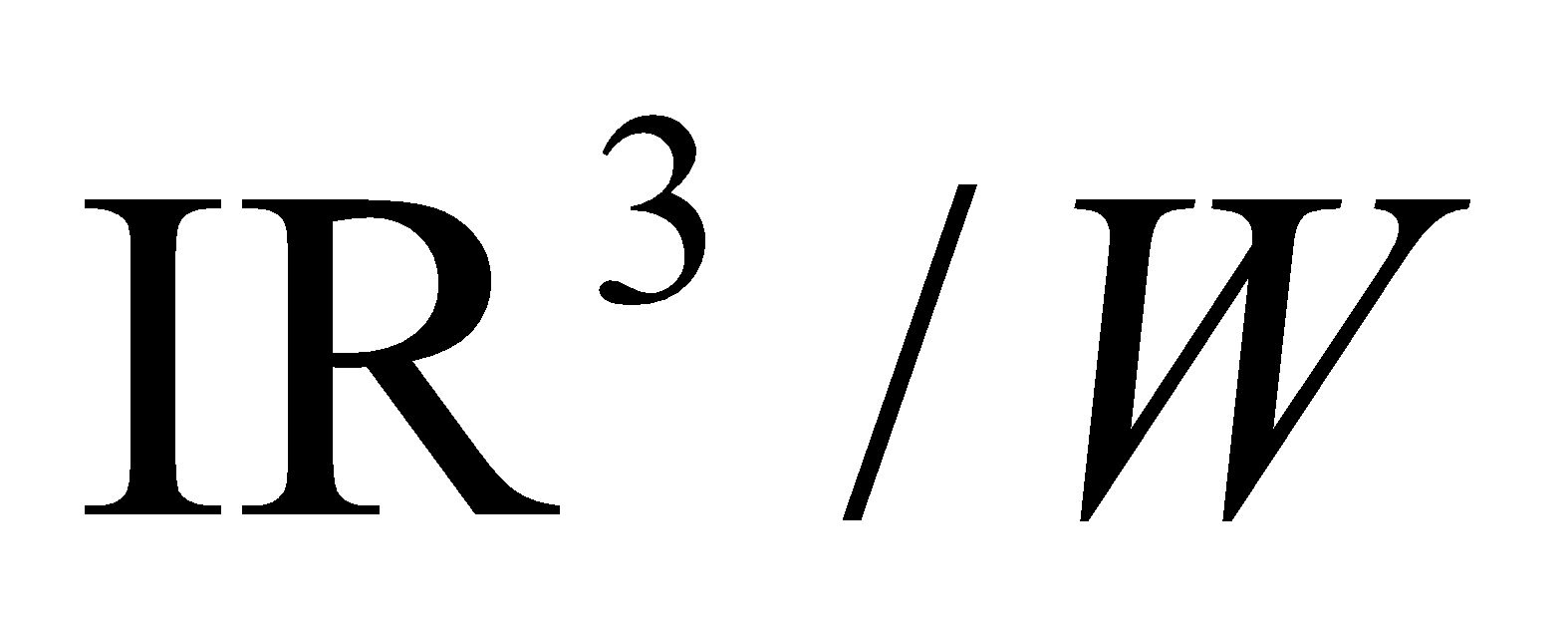. 3
5. Answer any two of the following:
1. Let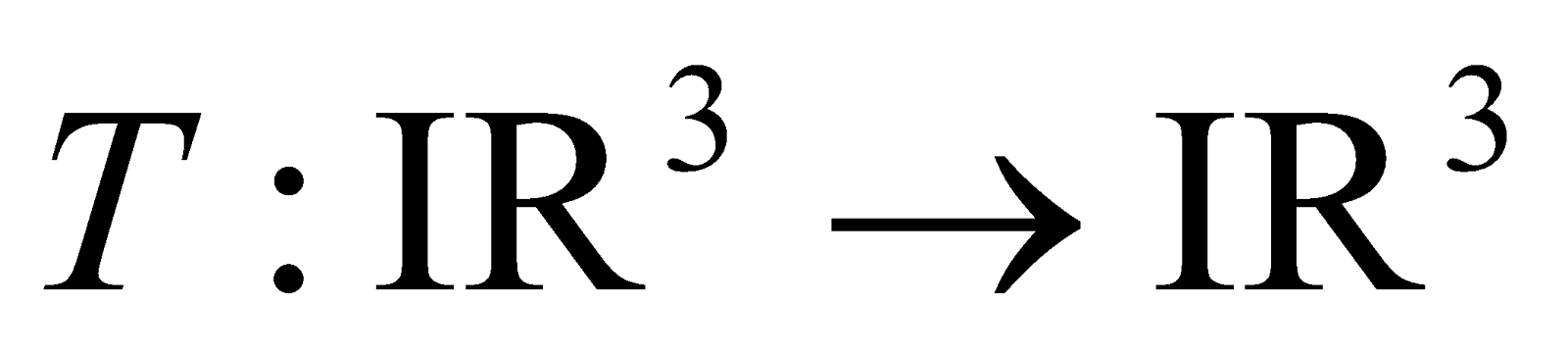be the mapping defined by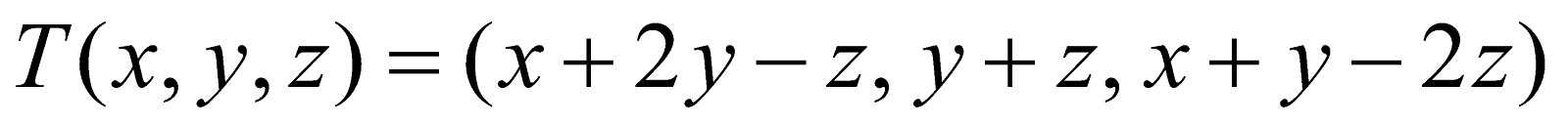Prove that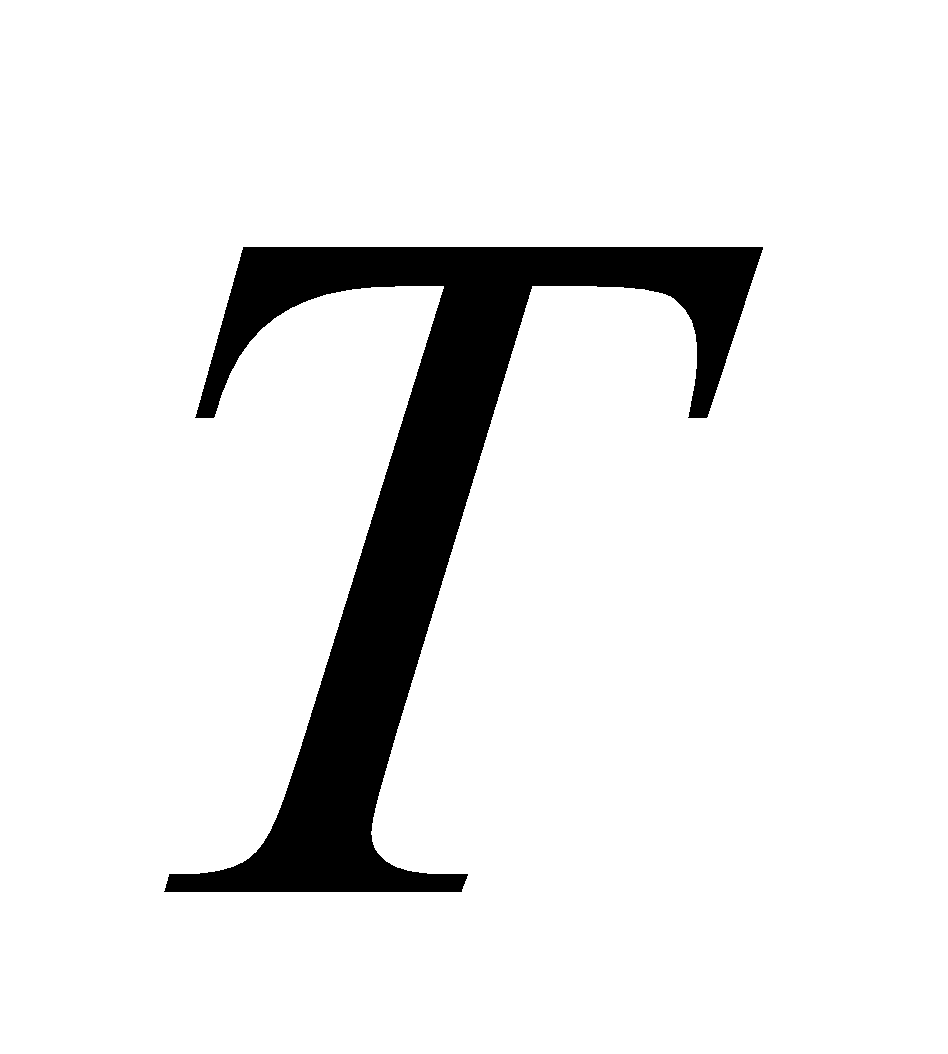is a linear mapping. Also find the basis and the dimension of the image of
2+3+1=6
1. Define a linear operator on a vector space with an example. Letbe a linear operator on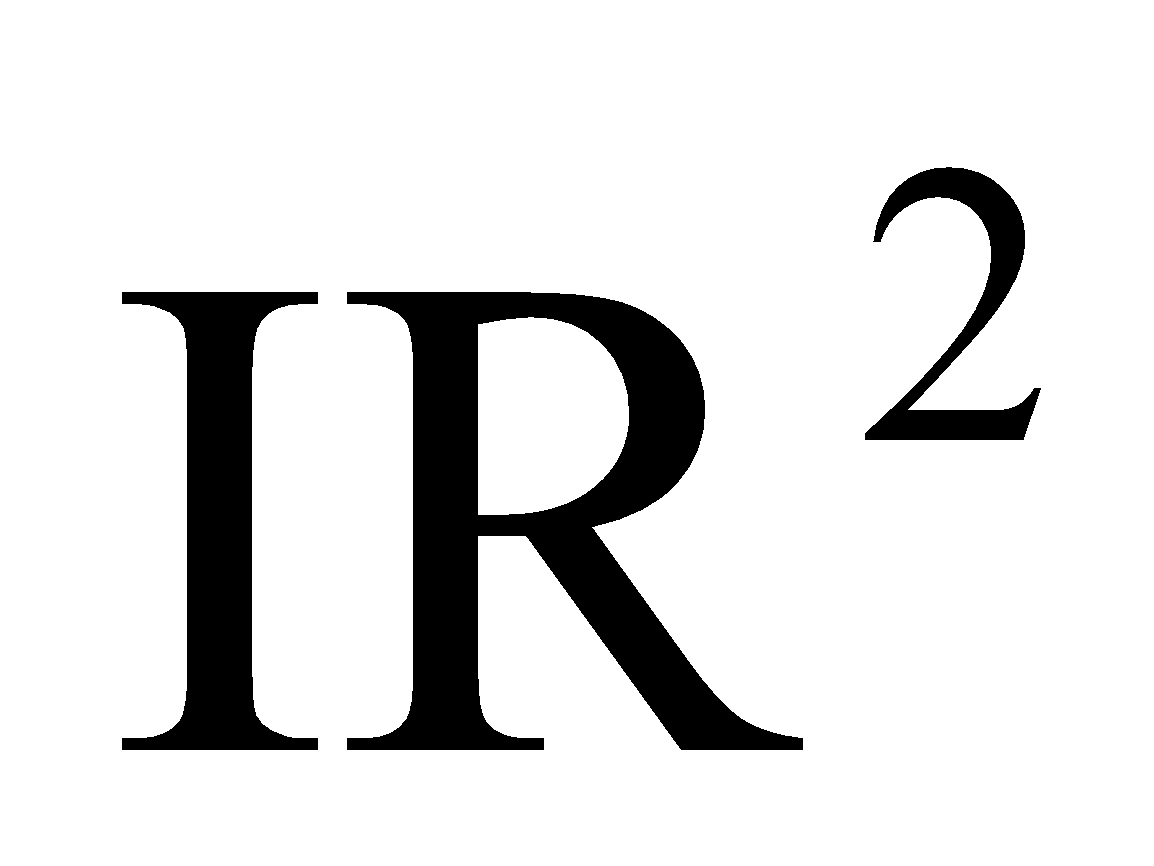given by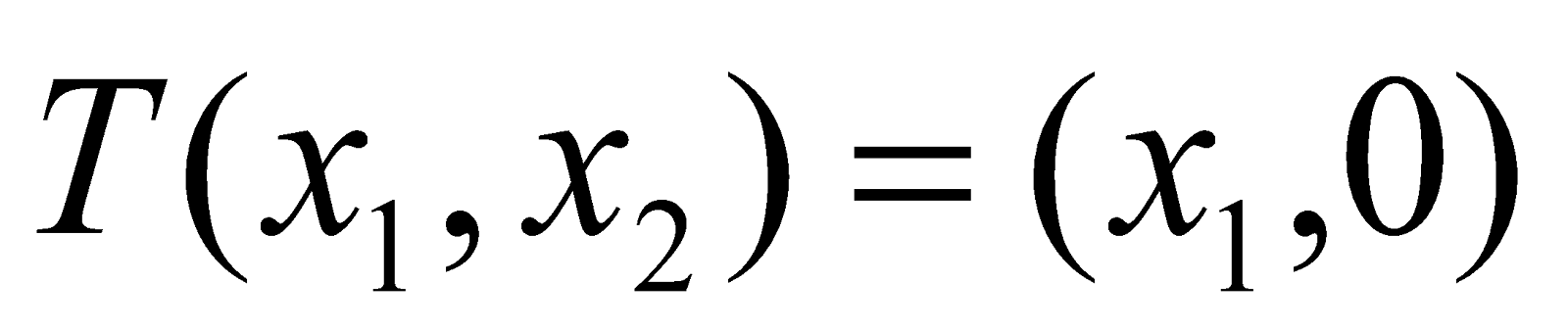. Find the matrix ofwith respect to the basis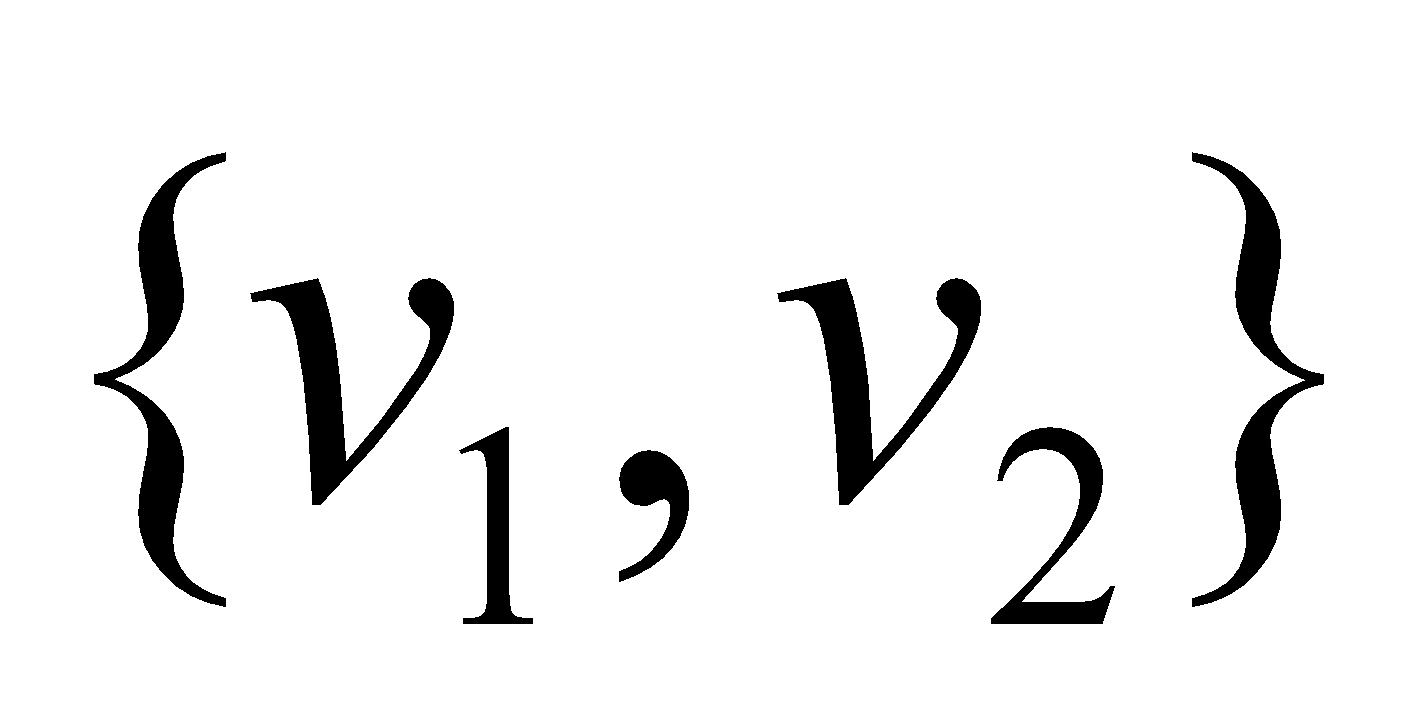where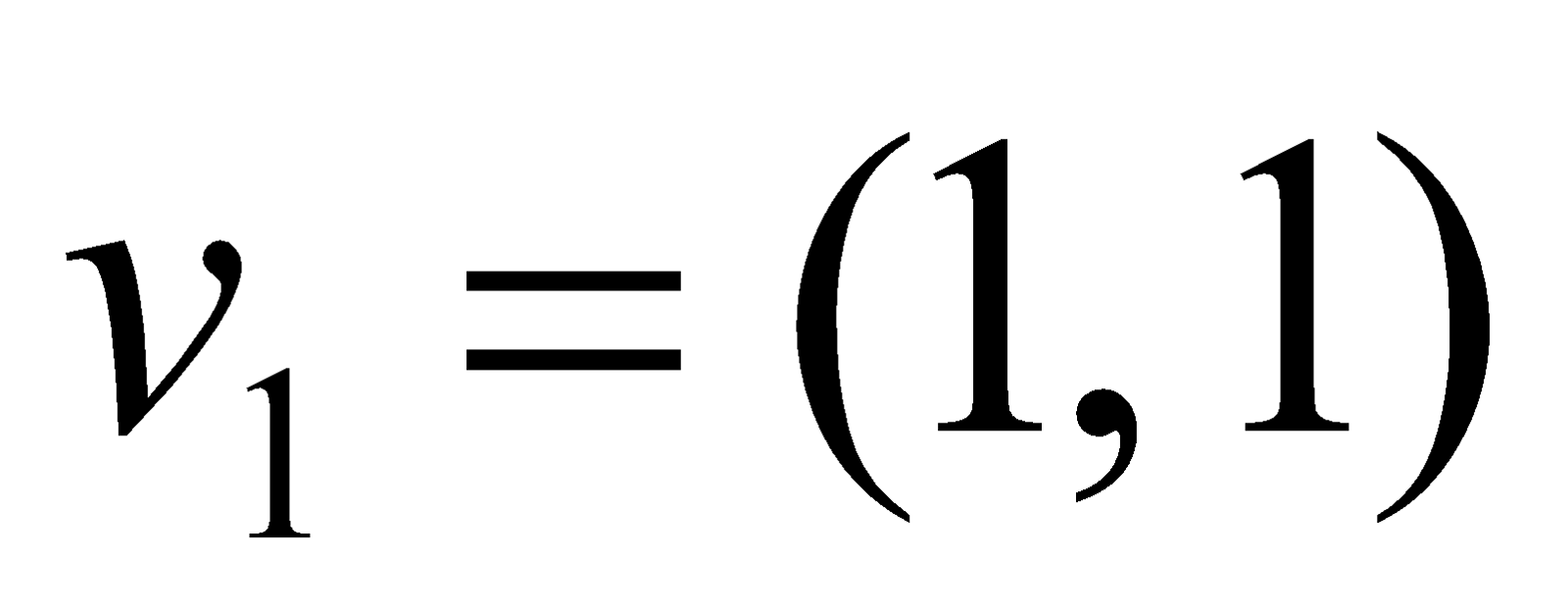and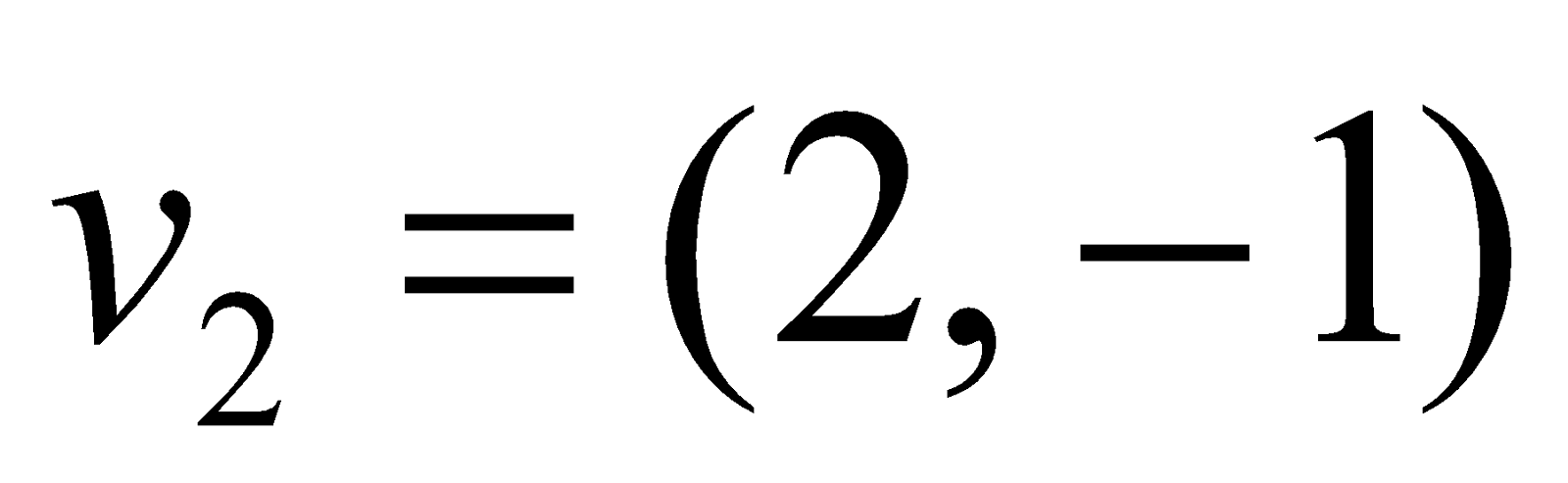. 2+4=6
2. Define isomorphism of vector spaces. Prove that the mapping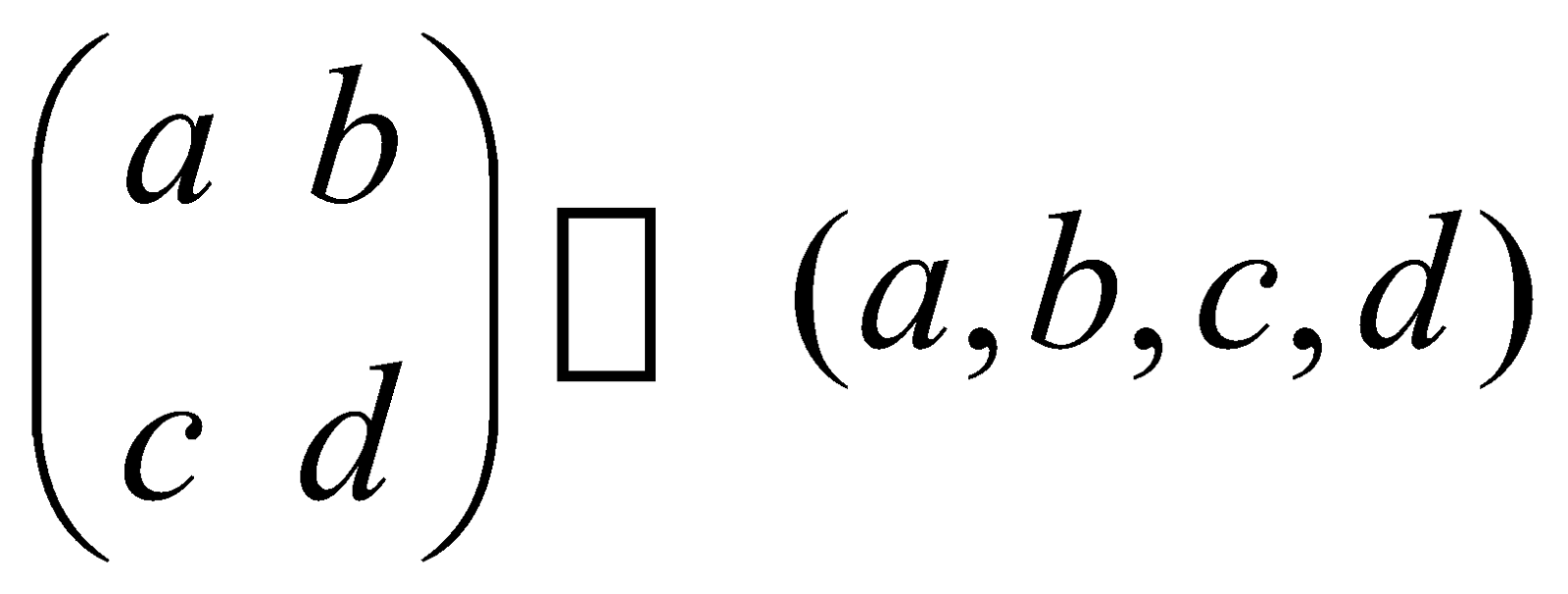fromtois an isomorphism.       2+4=6

GROUP – B
(Number Theory)
(Marks: 40)
6. (a) If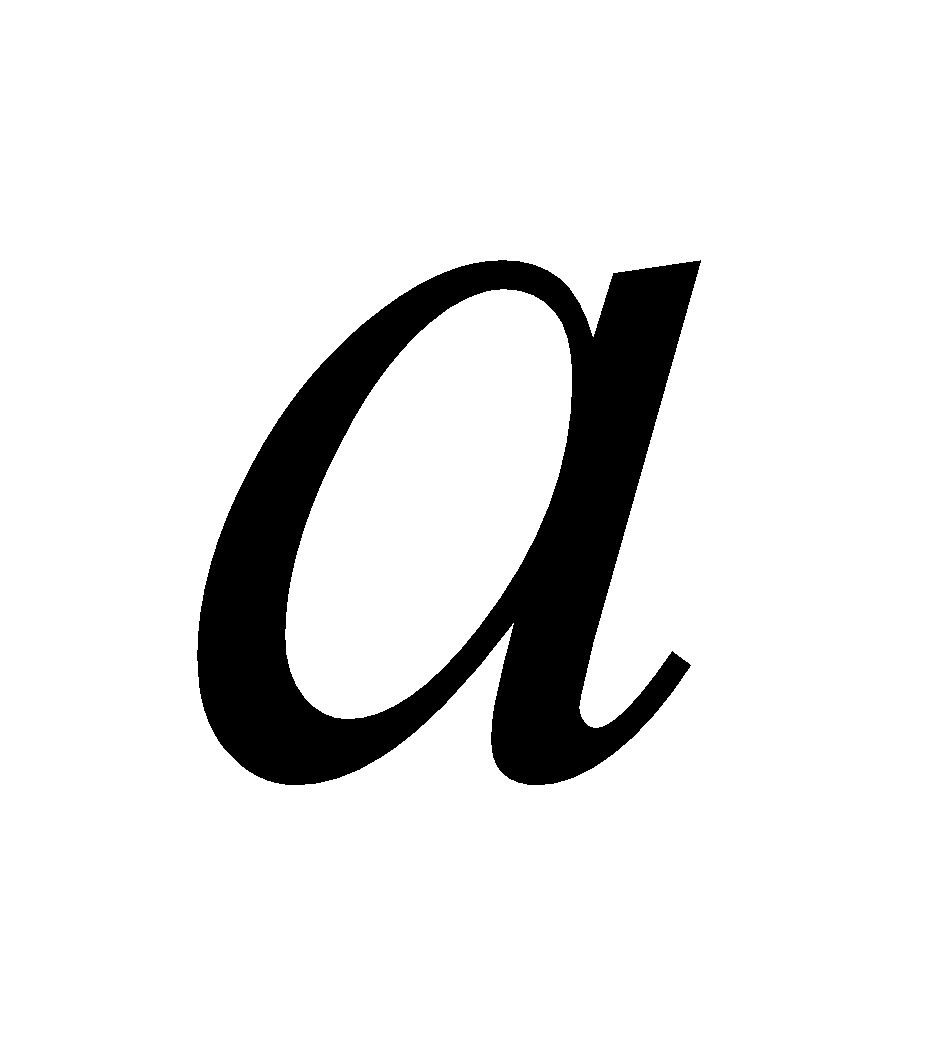and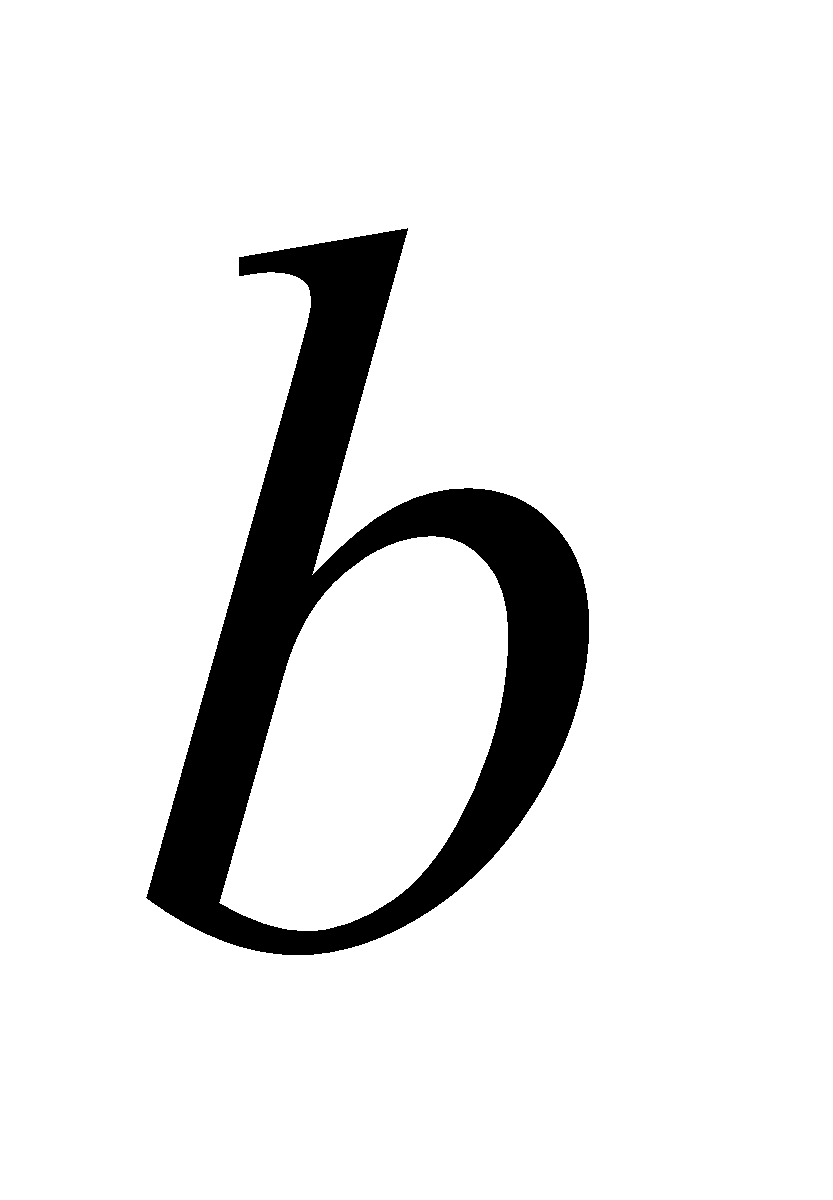are relatively prime, then prove that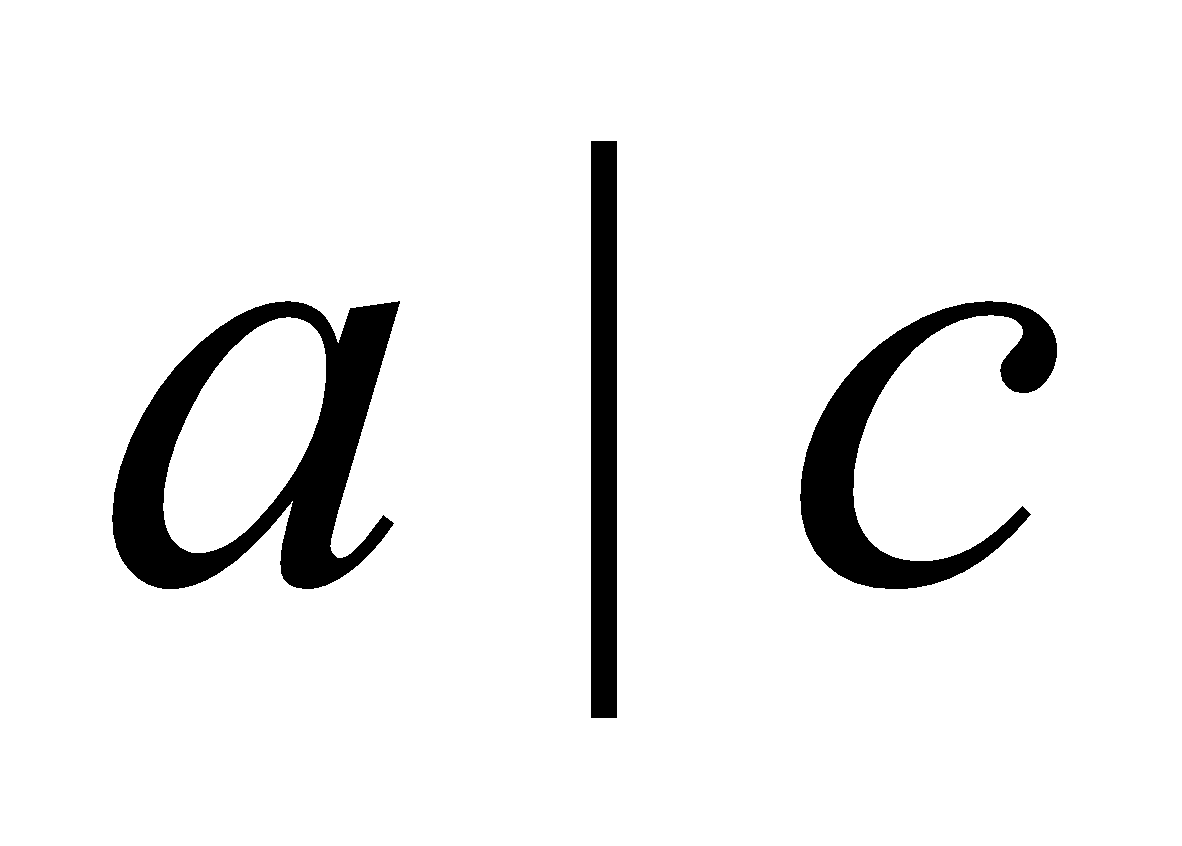and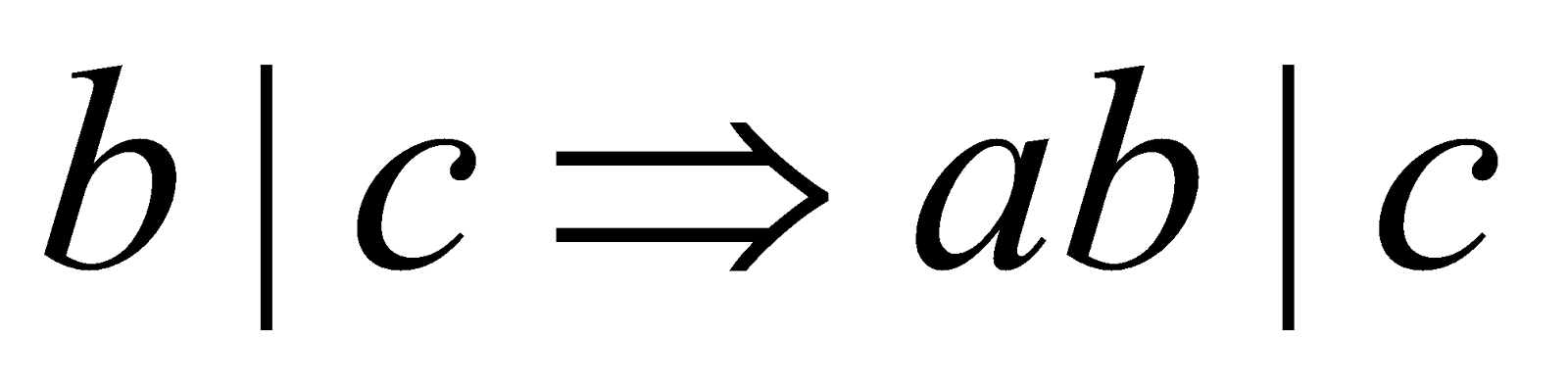. 2
(b) Find the integersand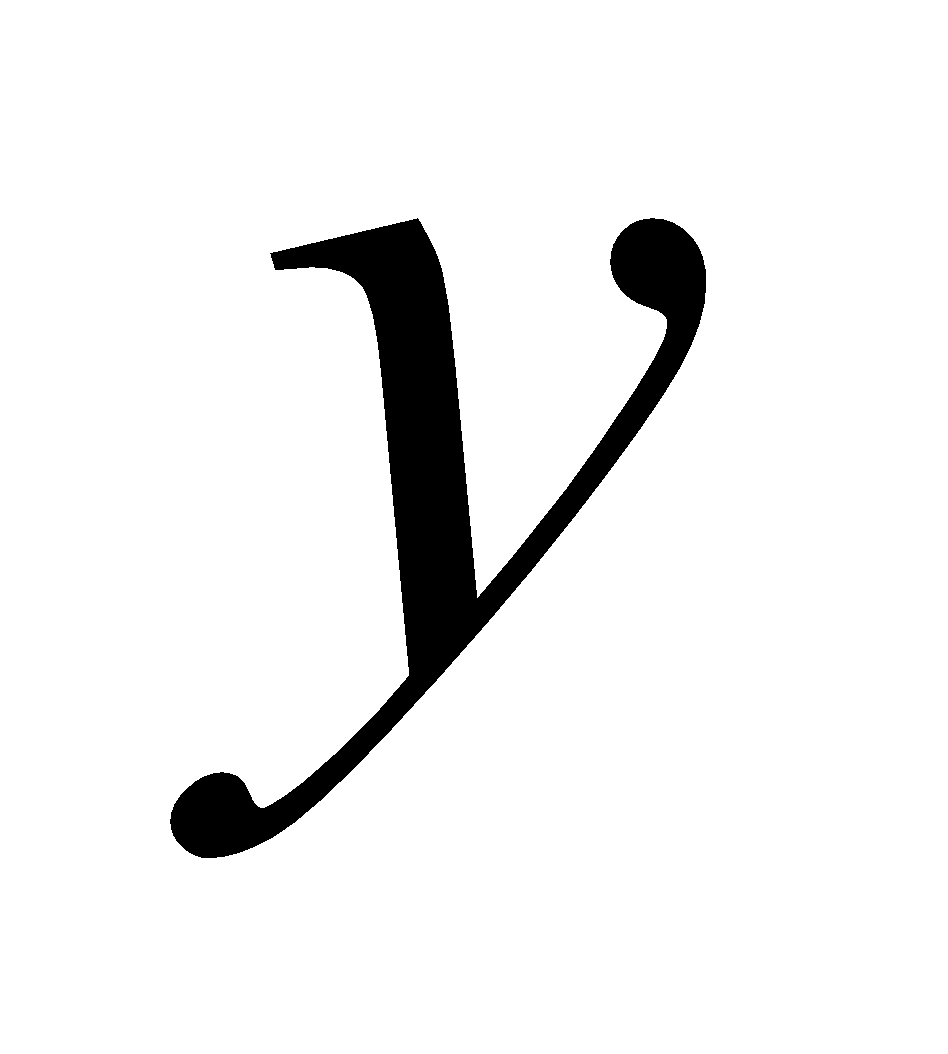such that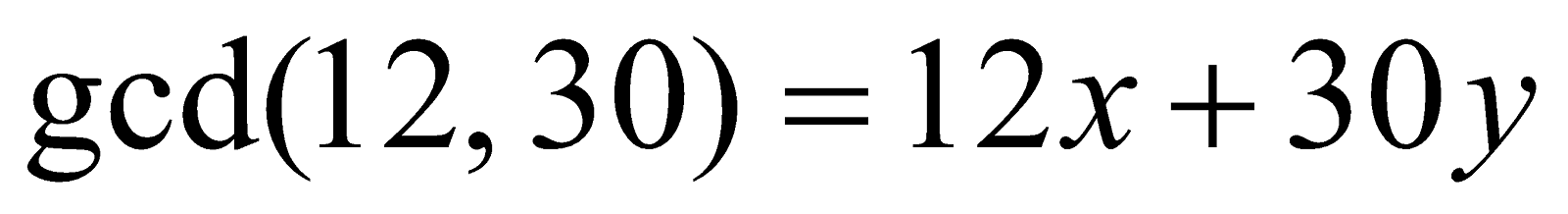. 2
(c) Use division algorithm to establish that the cube of any integer has one of the forms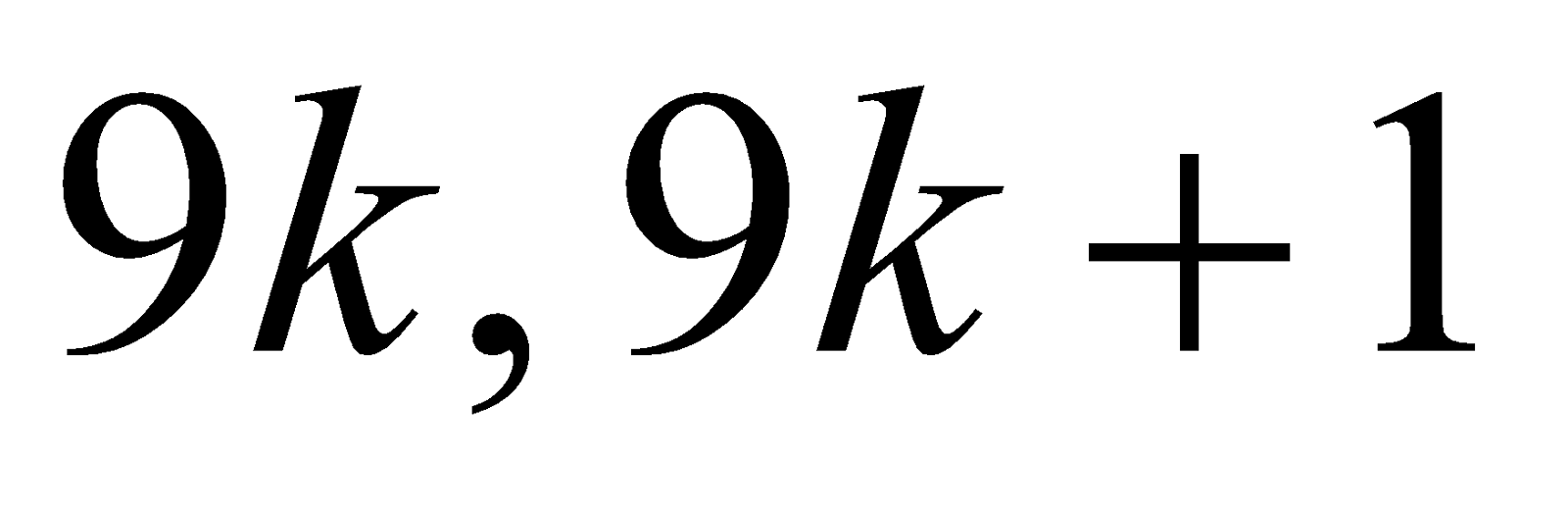or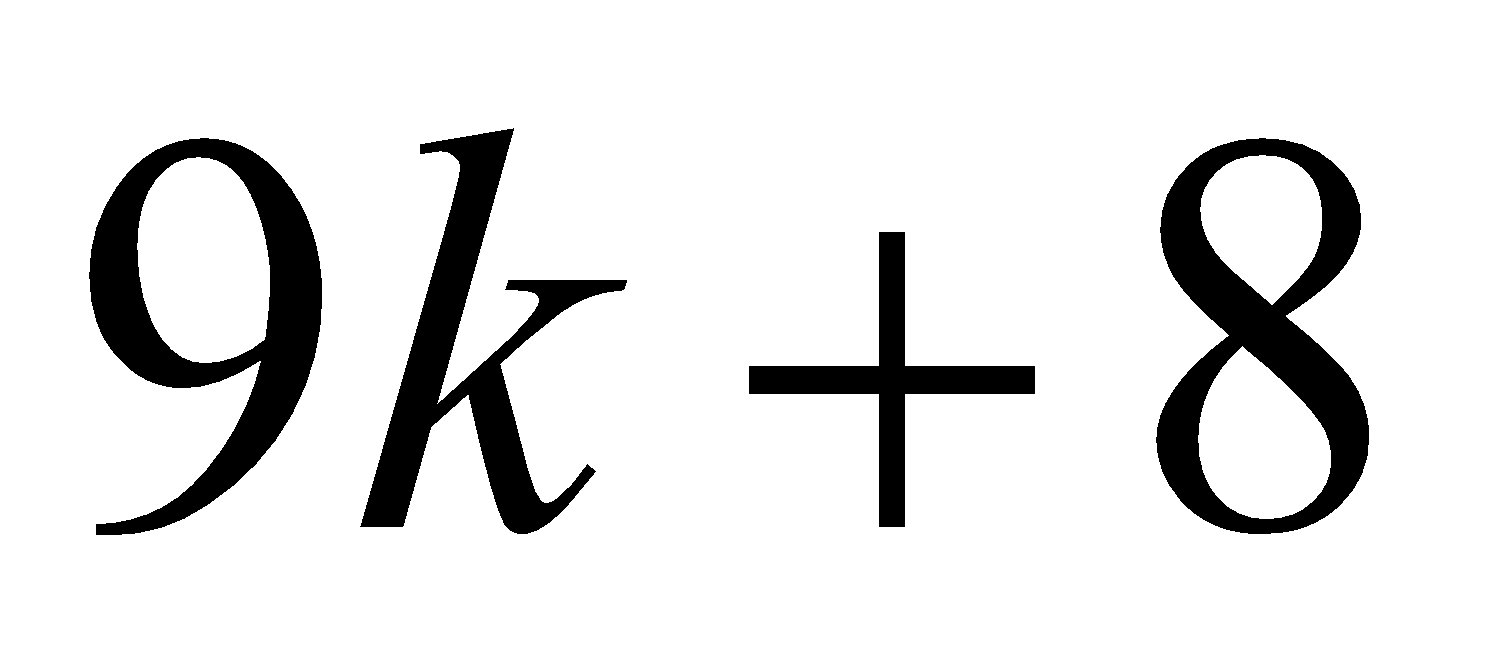.       3
7. (a) Find the number of divisors of 3 and 5 in between 500 and 1000. 3
(b) Find the highest power of 5 which is contained in 200!. 2
(c) Ifandare two integers such that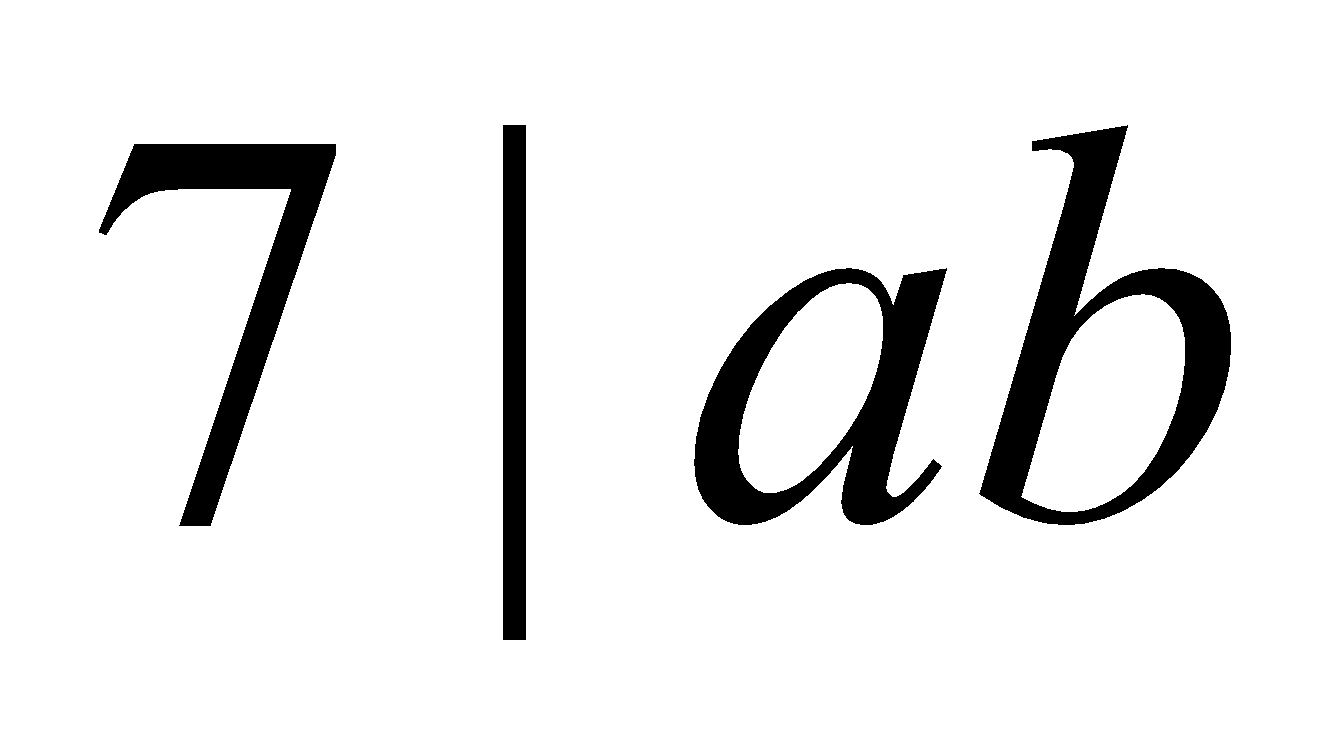, then show that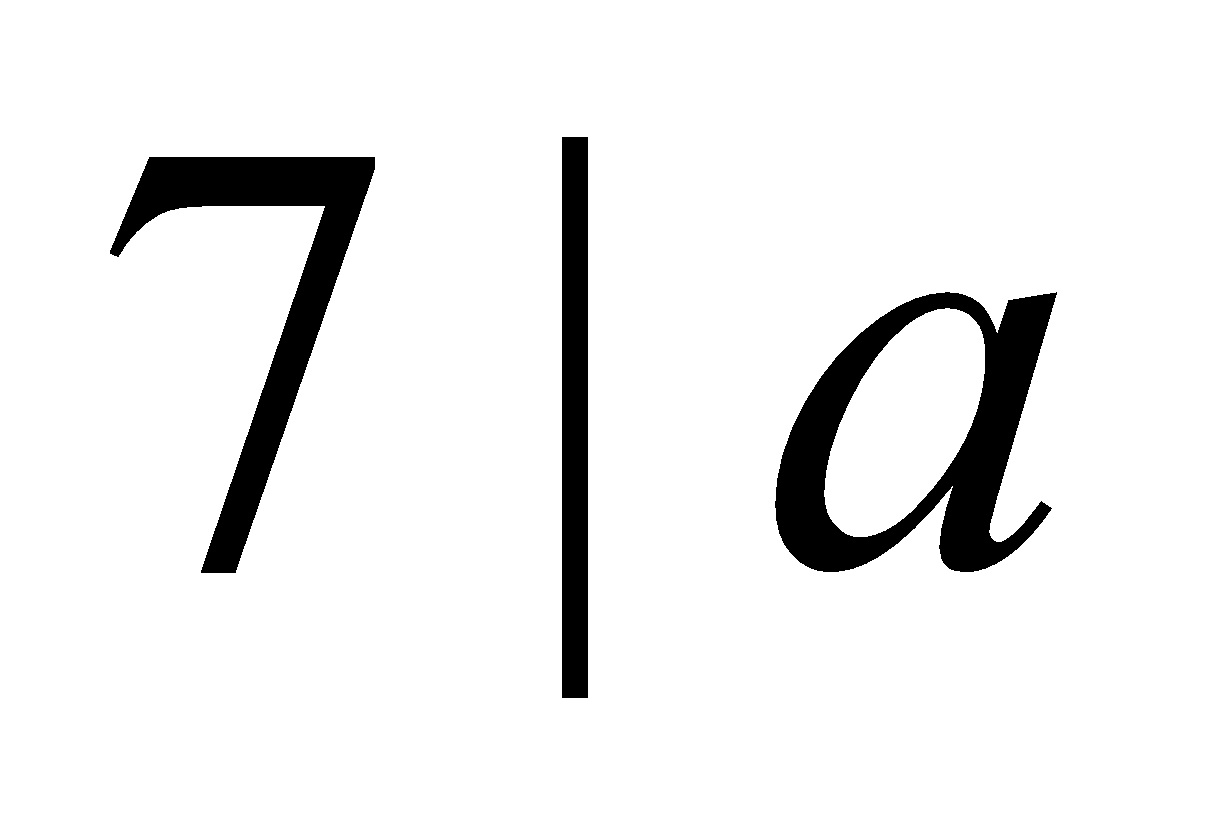or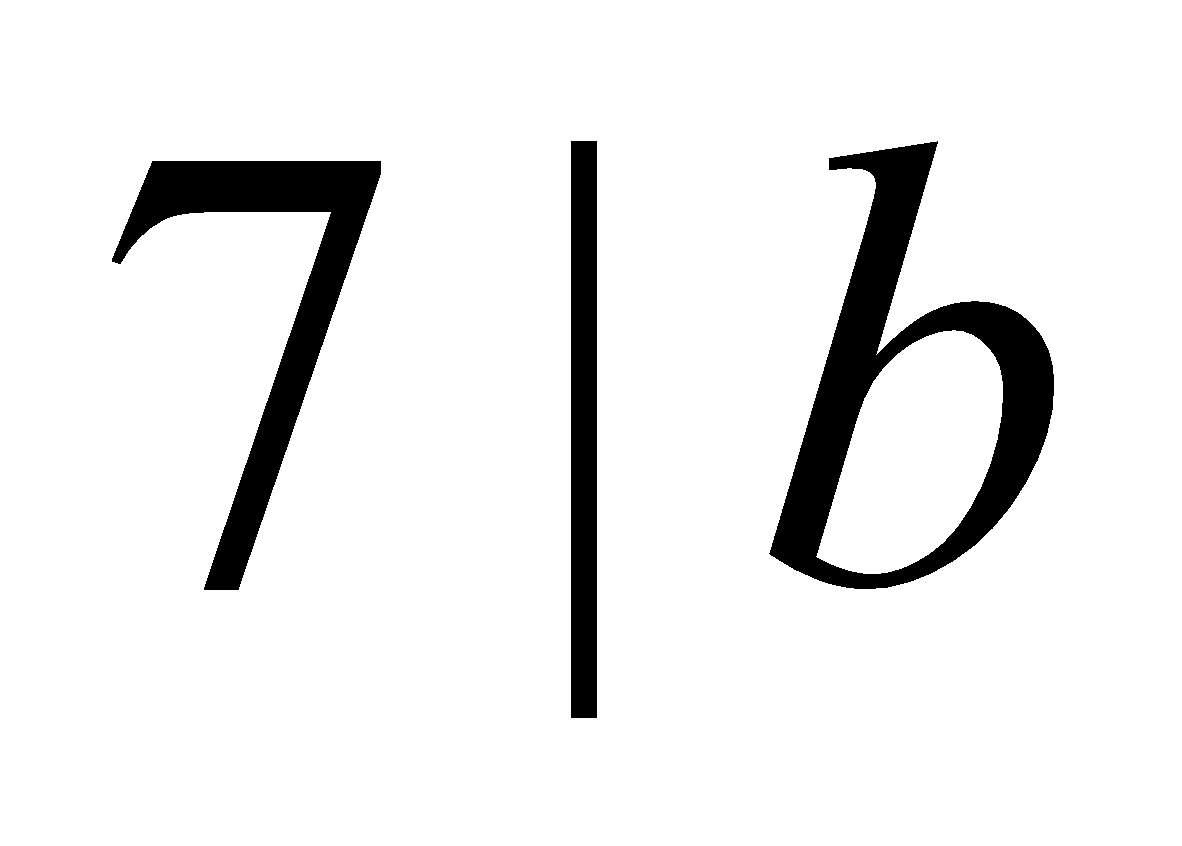. 3
8. (a) Write a reduced set of residues modulo 9. 1
(b) Find the remainder when the following sum is divided by 15: 3(c) Write the condition that is to be satisfied so that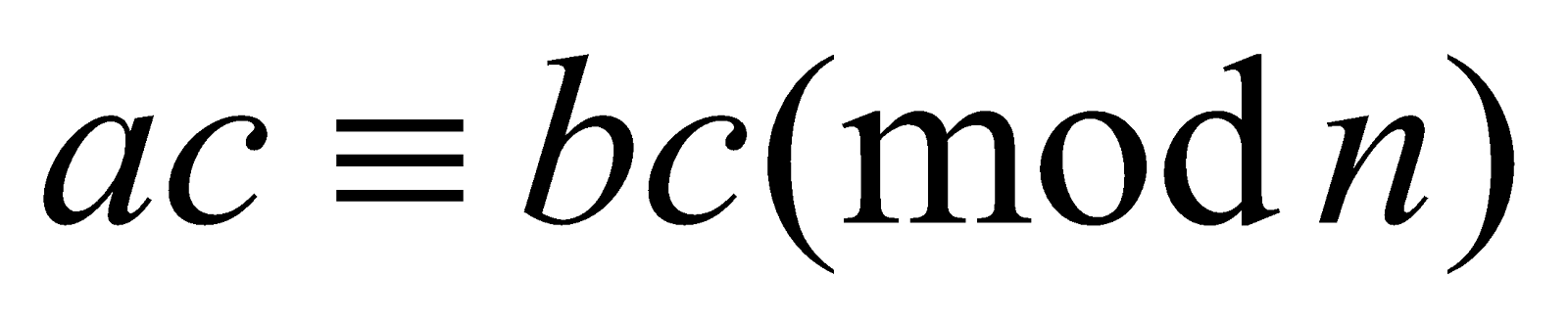gives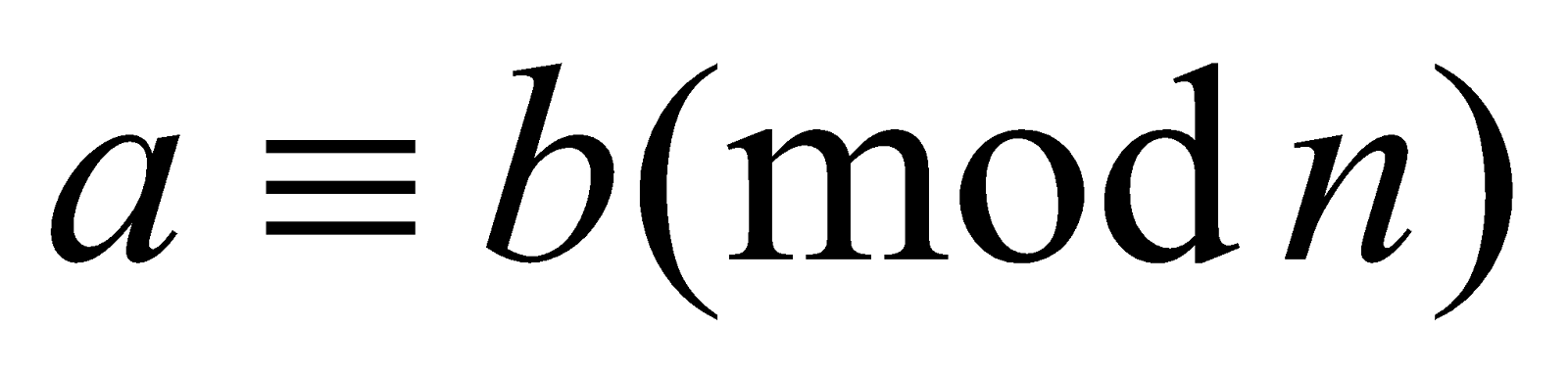. 1
(d) Find the remainder when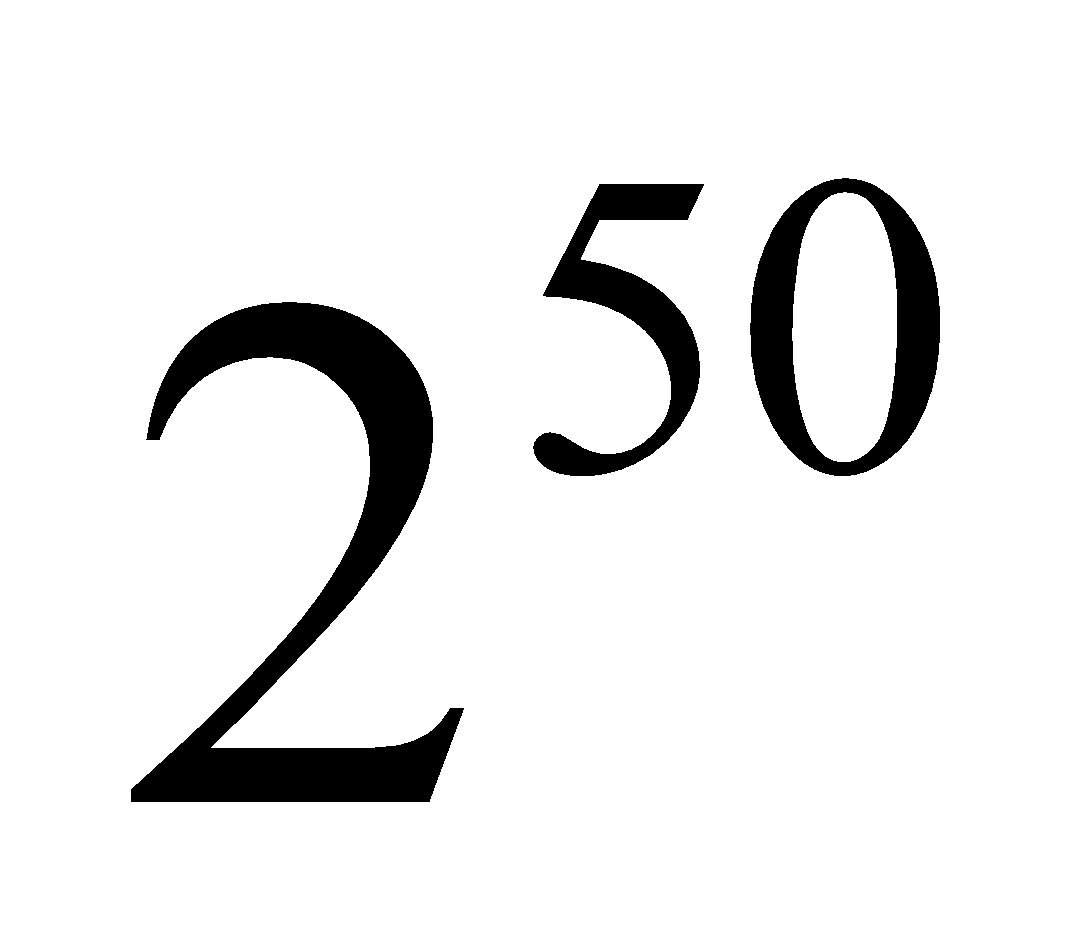is divided by 7. 2
(e) Does there exist any solution for the linear congruence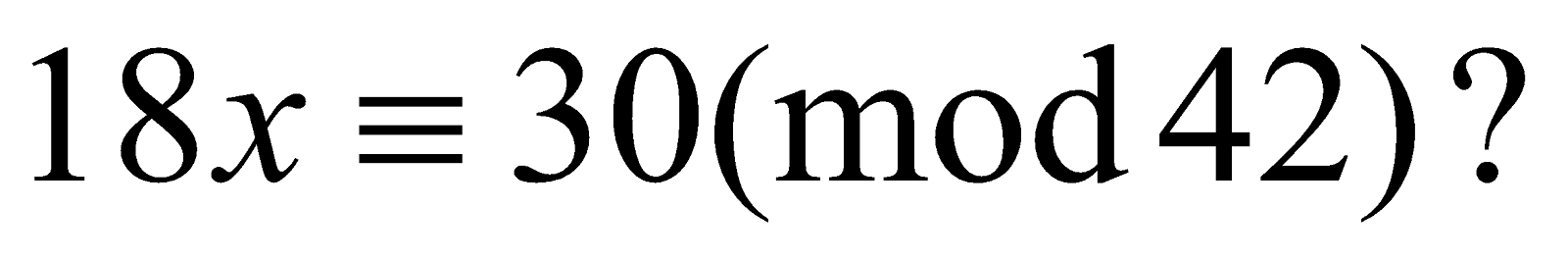If so, find all of them.                                                                                                                                                            1+4=5
(f) Solve: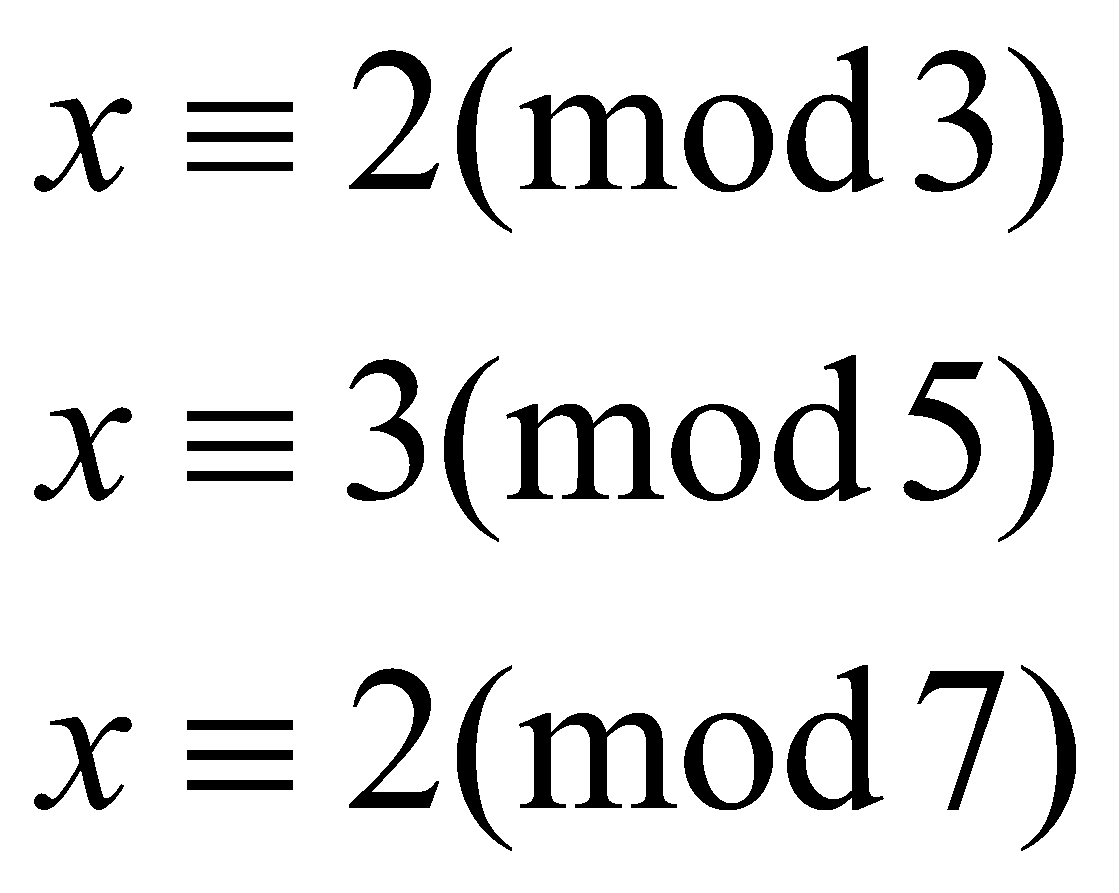3
9. (a) If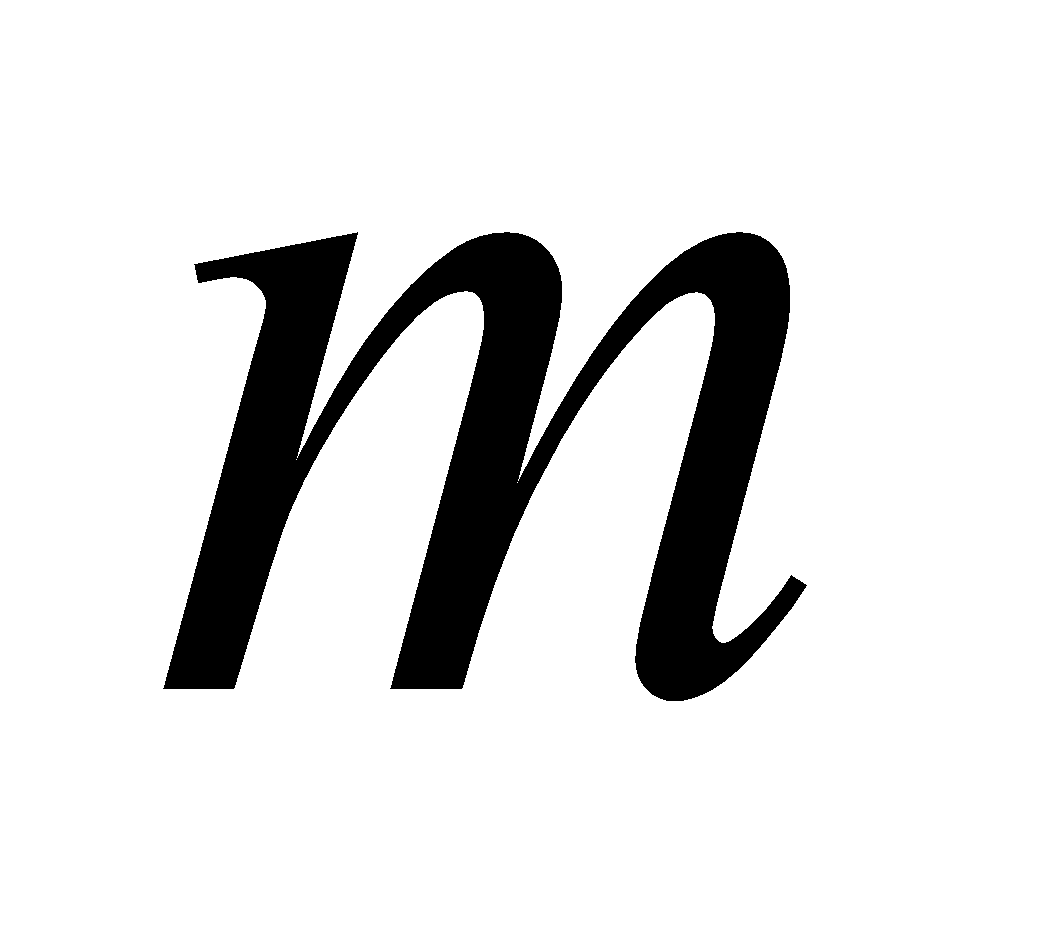and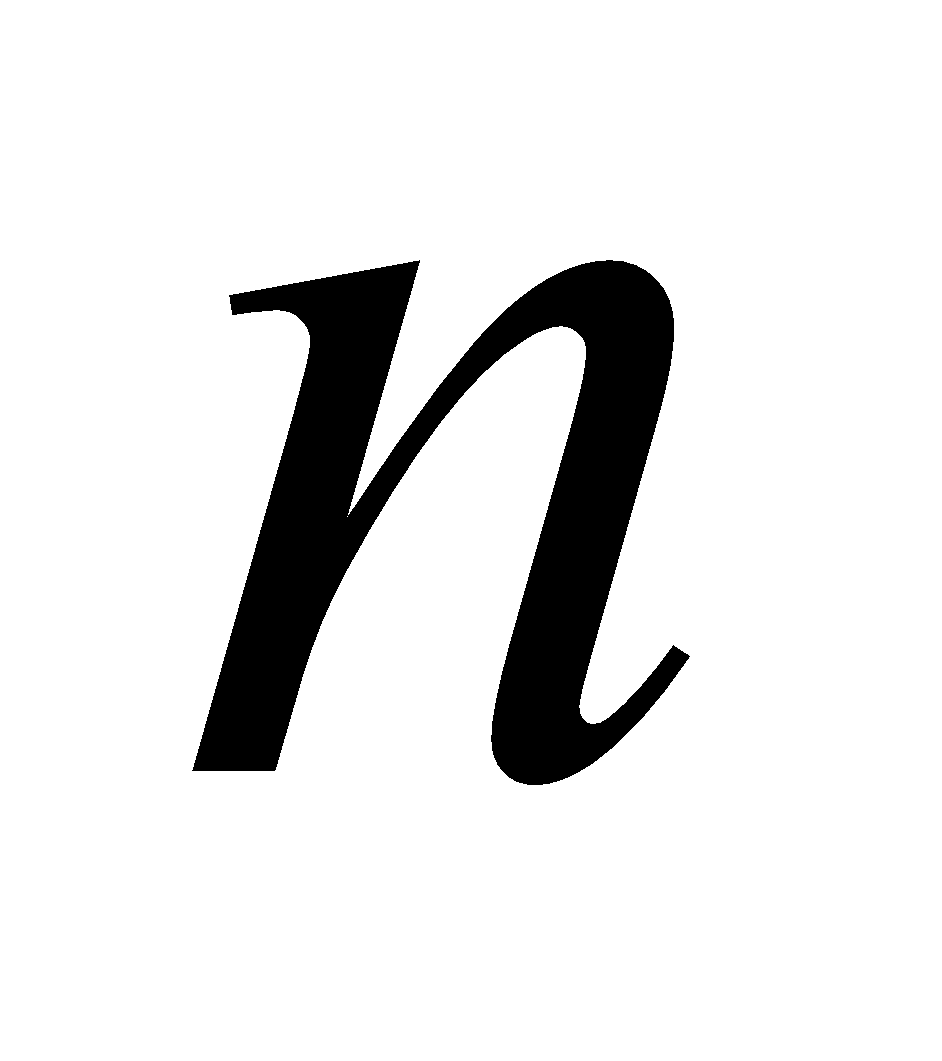are positive integers with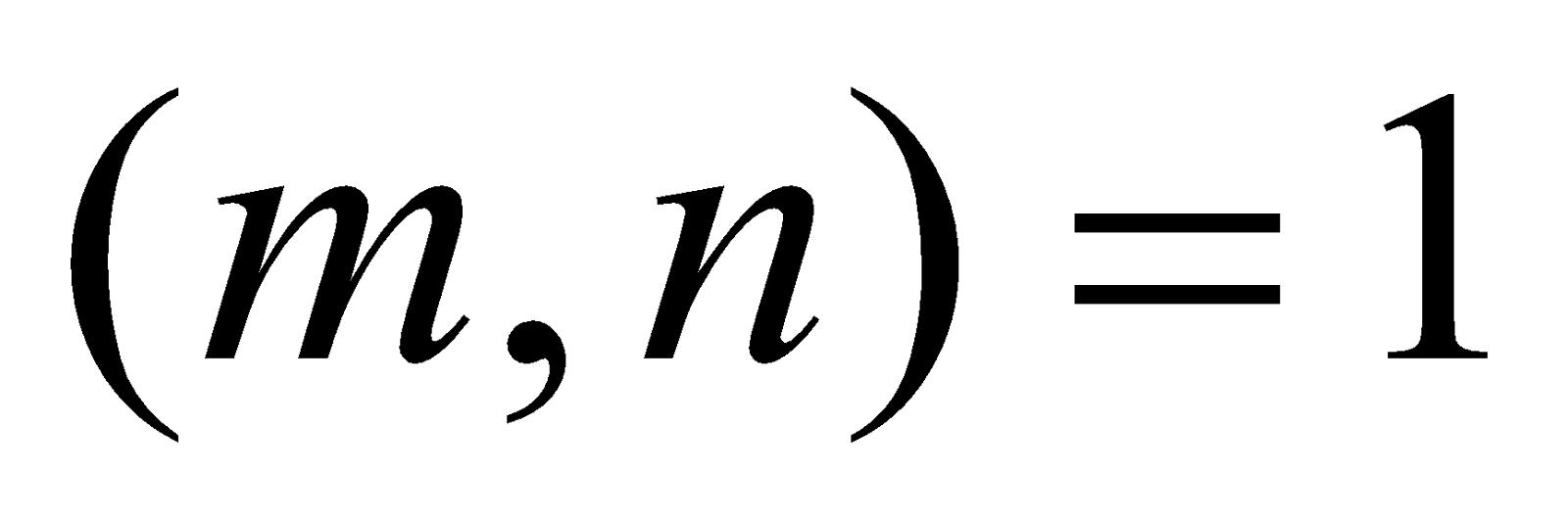, then prove that 2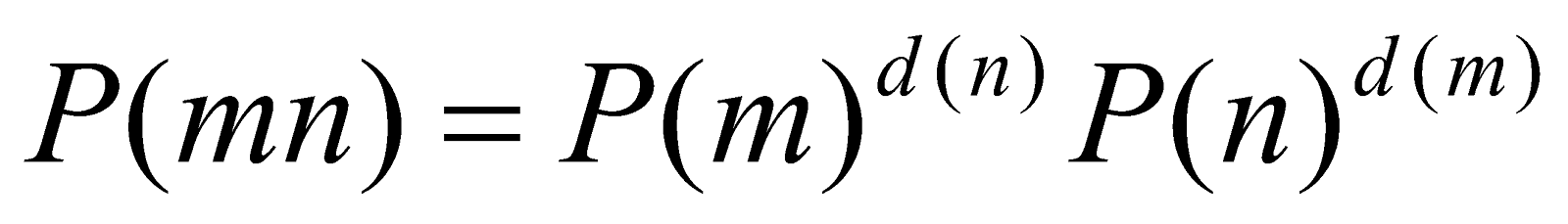(b) Find the value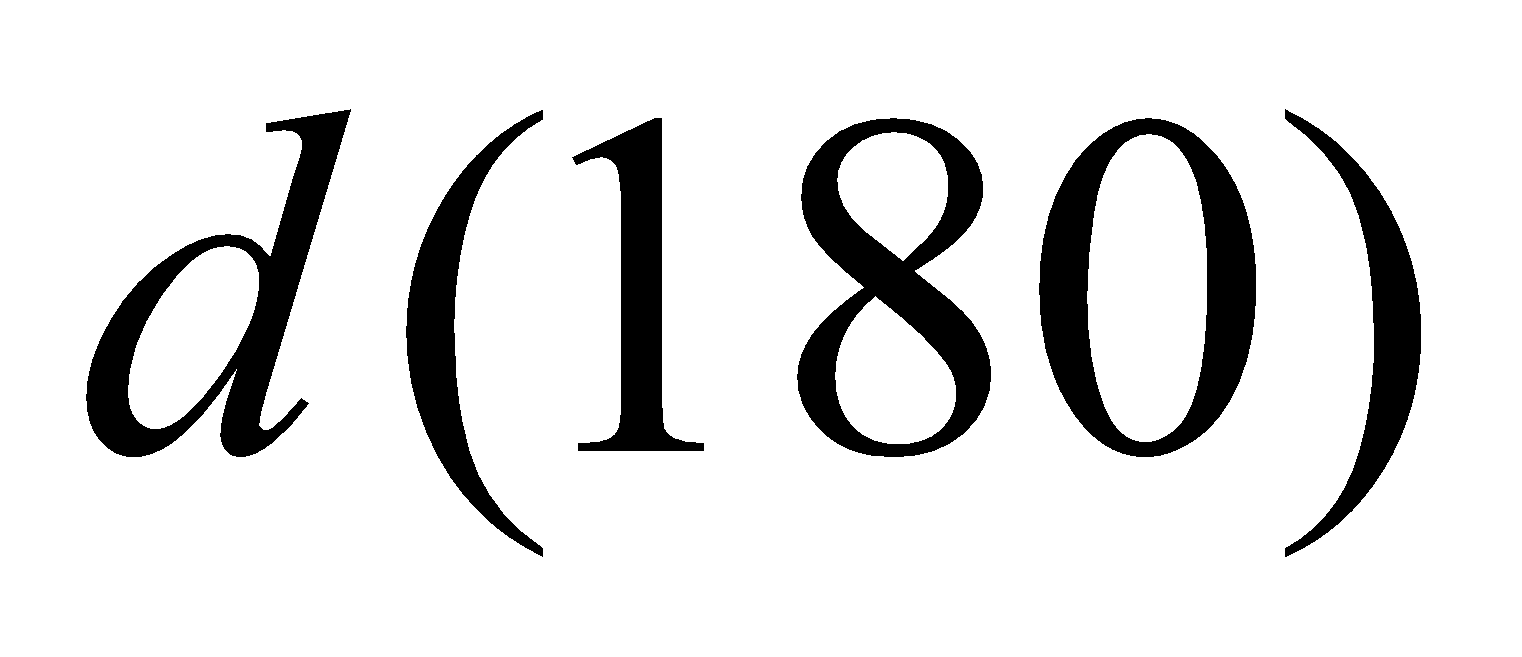. 2
(c) Show that if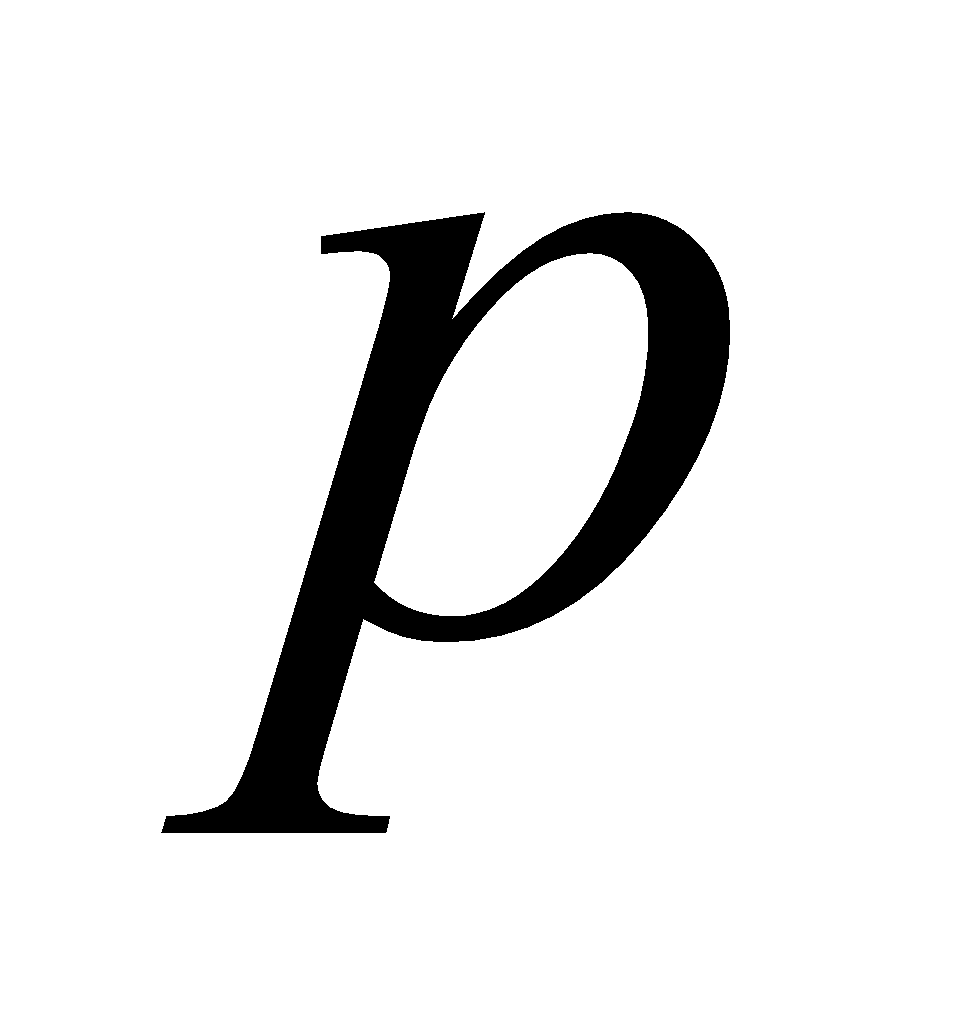is a prime number, then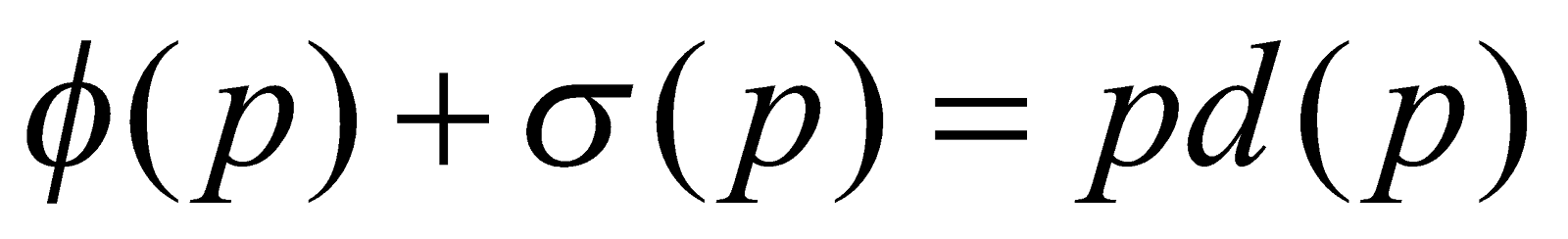2
(d) Define a multiplicative arithmetic function. Prove that the Mobius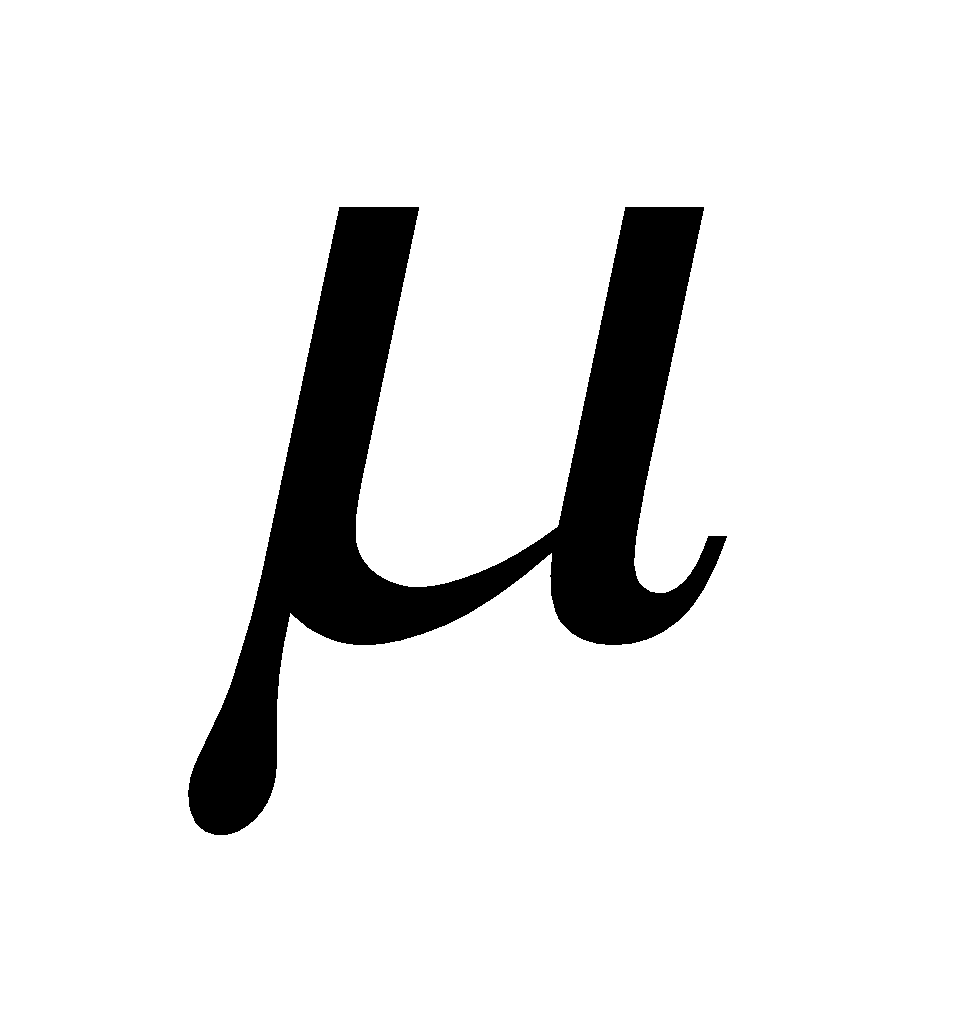-function is multiplicative function.    1+3=4
Or
Prove that there are infinitely many primes. 4

***